source("0_helpers.R")
opts_chunk$set(warning = F, message = F, error = T) all_surveys <- all_surveys %>% mutate(relationship_duration = duration_relationship_years + duration_relationship_month/12) ## mutate: new variable 'relationship_duration' with 187 unique values and 33% NA library(knitr) opts_chunk$set(fig.width = 9, fig.height = 7, cache = T, warning = F, message = F, cache = F, error = T)
library(kableExtra)

## Study flow chart

knitr::include_graphics("sfig_flow_chart_general_desire.png")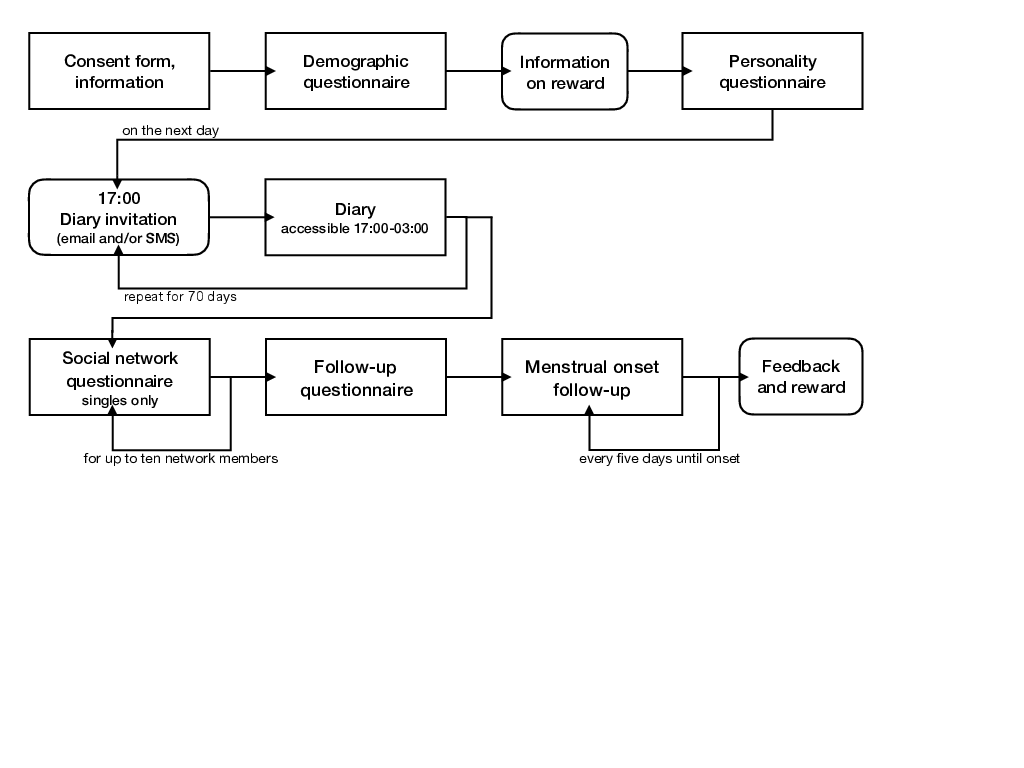Flow chart of the steps of the menstrual cycle diary study.

began_s1_demo = sum(!is.na(s1_demo$created)) ended_s1_demo = sum(!is.na(s1_demo$ended))
diary <- s1_filter %>% left_join(diary, by = c('session', 'short'), suffix = c("_filter", ""))
ended_pre = diary %>% filter(!is.na(ended_initial)) %>% ungroup() %>% summarise(n = n_distinct(session)) %>% pull(n)
diary %>% group_by(gets_paid) %>% summarise(n = sum(!is.na(ended_initial[!duplicated(session)]), n = n_distinct(session)))
## # A tibble: 2 x 2
##   gets_paid     n
##   <chr>     <int>
## 1 0          1893
## 2 1          1087
at_least_one_diary_entry = diary %>% group_by(session) %>% summarise(n = n_nonmissing(ended_diary)) %>% filter(n >= 1) %>% nrow()
at_least_20_diary_entries = diary %>% group_by(session) %>% summarise(n = n_nonmissing(ended_diary)) %>% filter(n >= 20) %>% nrow()
at_least_50_diary_entries = diary %>% group_by(session) %>% summarise(n = n_nonmissing(ended_diary)) %>% filter(n >= 50) %>% nrow()

in_relationship = sum(s1_demo$hetero_relationship, na.rm = T) singles = sum(! s1_demo$hetero_relationship, na.rm = T)

at_least_one_diary_entry = s3_daily %>% group_by(session) %>% summarise(n = n_nonmissing(ended)) %>% filter(n >= 1) %>% nrow()
at_least_20_diary_entries = s3_daily %>% group_by(session) %>% summarise(n = n_nonmissing(ended)) %>% filter(n >= 20) %>% nrow()
at_least_50_diary_entries = s3_daily %>% group_by(session) %>% summarise(n = n_nonmissing(ended)) %>% filter(n >= 50) %>% nrow()

mean_diary_entries <- s3_daily %>% group_by(session) %>% summarise(n = n_nonmissing(ended)) %>% summarise(mean(n))

mean_diary_entries_by_payment <- s1_filter %>%
select(-ended) %>% left_join(s3_daily, by = 'session') %>%
group_by(gets_paid, session) %>%
summarise(n = n_nonmissing(ended)) %>%
filter(n > 0) %>%
group_by(gets_paid) %>%
summarise(responses = mean(n), n = n_distinct(session))

response_rate_over_time <- diary %>%
filter(day_number >= 0, day_number < 70) %>%
left_join(s1_filter, by = 'session', suffix = c("", "_filter")) %>%
group_by(gets_paid) %>%
mutate(
n = n_distinct(session)
) %>%
group_by(gets_paid, day_number) %>%
summarise(
n = unique(n),
entries = n_nonmissing(ended_diary),
rr = entries / unique(n))

ggplot(response_rate_over_time, aes(day_number, rr, colour = gets_paid)) + geom_point() +
ggtitle("Response rate over time", "for people who started the diary") + ylim(0,1)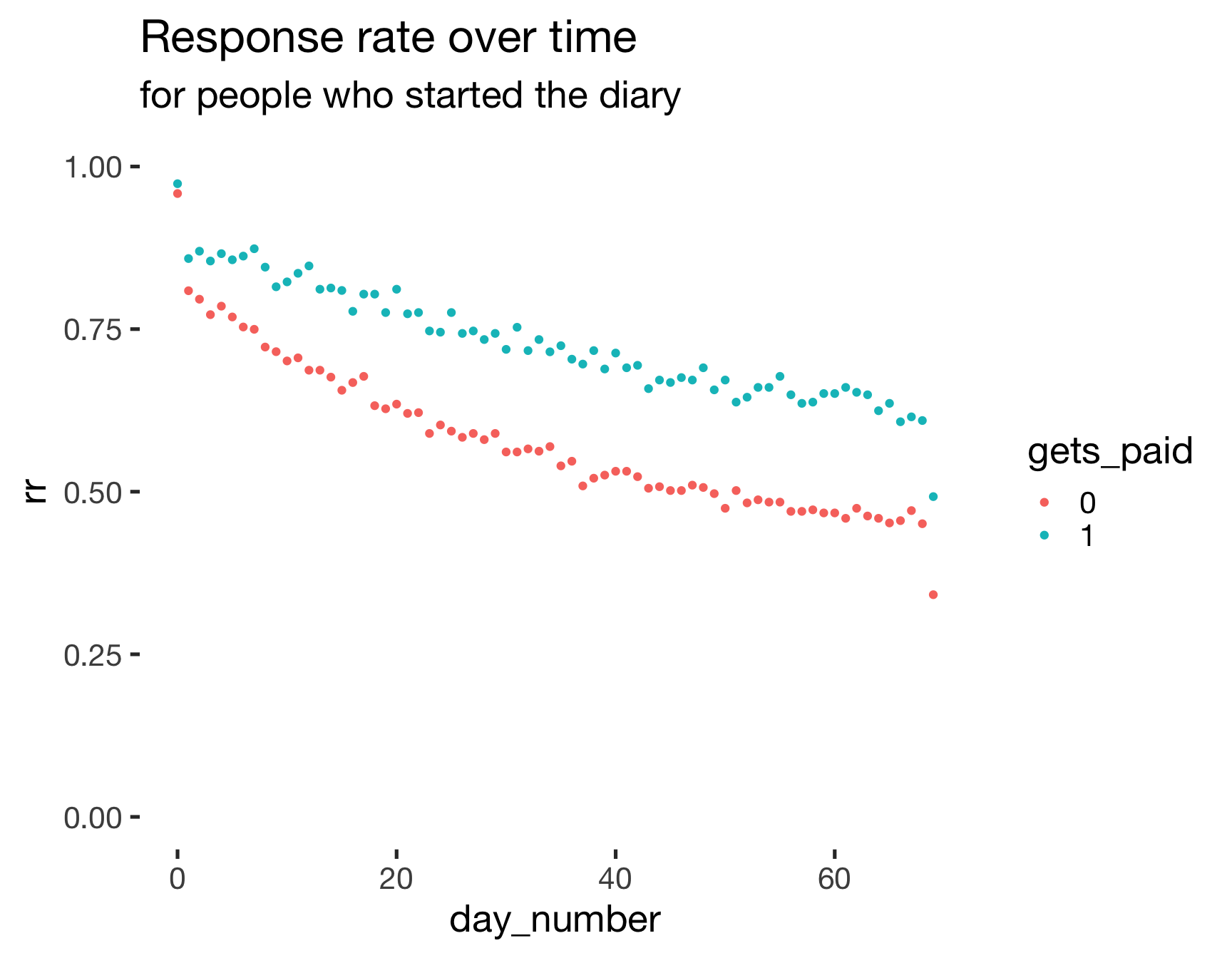mean_diary_entries_finishers <- s4_followup %>% select(-ended) %>% left_join(s3_daily, by = 'session') %>% group_by(session) %>% summarise(n = n_nonmissing(ended)) %>% summarise(mean(n))

response_rate_over_time <- s4_followup %>% select(-ended) %>%
left_join(diary %>% select(session, day_number, created_diary, ended_diary)) %>% filter(day_number >= 0, day_number < 70) %>%
left_join(s1_filter, by = 'session', suffix = c("", "_filter")) %>%
group_by(gets_paid) %>%
mutate(
n = n_distinct(session)
) %>%
group_by(gets_paid, day_number) %>%
summarise(
entries = n_nonmissing(ended_diary),
rr = entries / unique(n))

ggplot(response_rate_over_time, aes(day_number, rr, colour = gets_paid)) +
geom_point() +
ggtitle("Response rate over time", "for people who finished the diary") + ylim(0,1)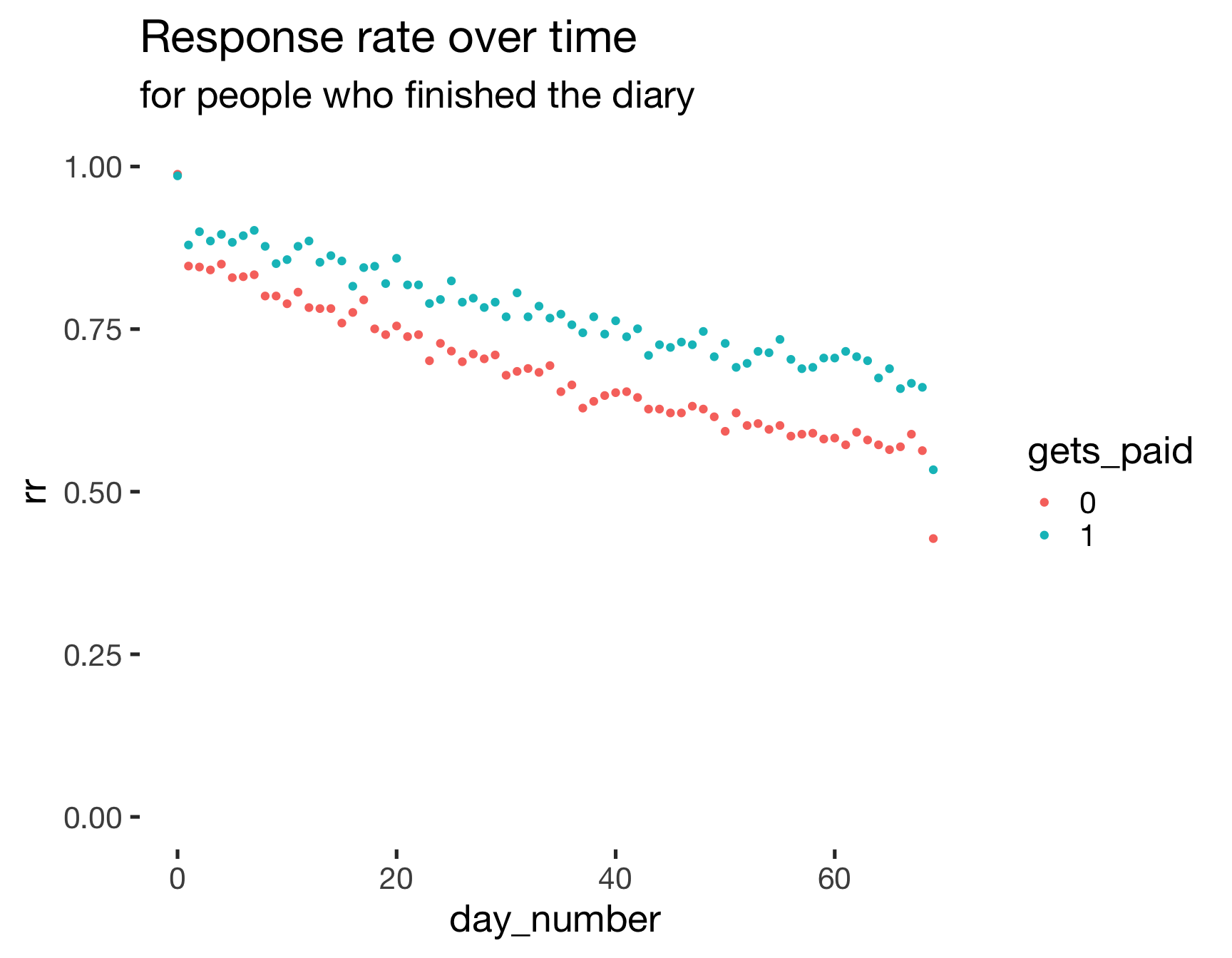response_rate_over_time <- diary %>%
# I am leaving in days that we excluded post-hoc (e.g., because fertility was not estimable, to show the real response rate, irrespective of diary-data-based exclusions)
filter(reasons_for_exclusion == reasons_for_exclusion_diary) %>%
select(session, day_number, created_diary, ended_diary) %>%
filter(day_number >= 0, day_number < 70) %>%
left_join(s1_filter, by = 'session', suffix = c("", "_filter")) %>%
group_by(gets_paid) %>%
mutate(
n = n_distinct(session)
) %>%
group_by(gets_paid, day_number) %>%
summarise(
n = unique(n),
entries = n_nonmissing(ended_diary),
rr = entries / unique(n))

ggplot(response_rate_over_time, aes(day_number, rr, colour = gets_paid)) +
geom_point() +
ggtitle("Response rate over time", "for included subsample") + ylim(0,1)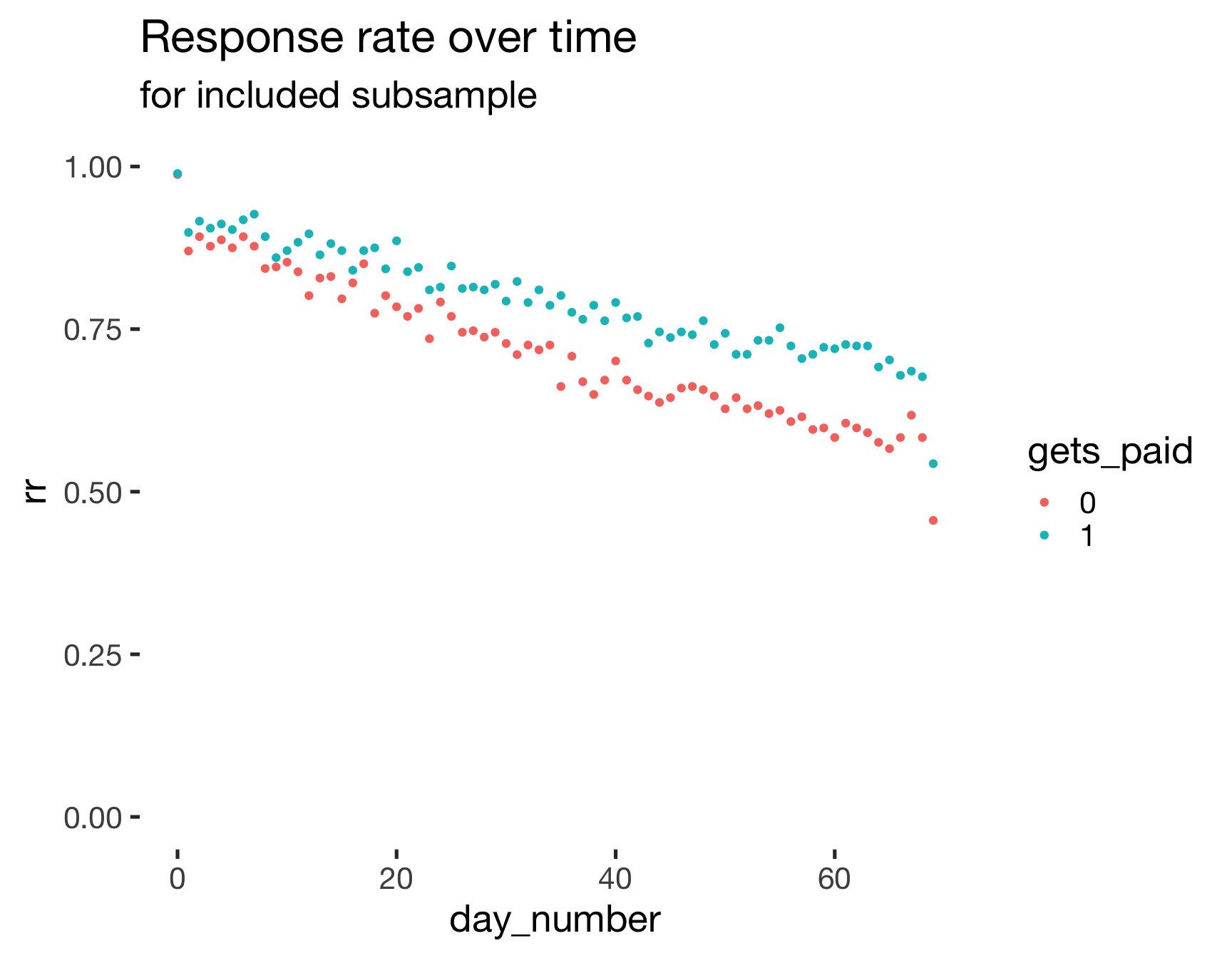nsmt2d_over_time <- s4_followup %>% select(-ended) %>%
left_join(diary %>% select(session, day_number, never_skipped_more_than_2, created_diary, ended_diary)) %>%
left_join(s1_filter, by = 'session', suffix = c("", "_filter")) %>%
left_join(mean_diary_entries_by_payment) %>%
group_by(gets_paid, day_number) %>%
summarise(entries = sum(as.numeric(never_skipped_more_than_2), na.rm = T),
rr = entries / unique(n))

ggplot(nsmt2d_over_time, aes(day_number, rr, colour = gets_paid)) +
geom_point() +
ggtitle("Percentage who never skipped more than two days over time", "for people who finished the diary") + ylim(0,1)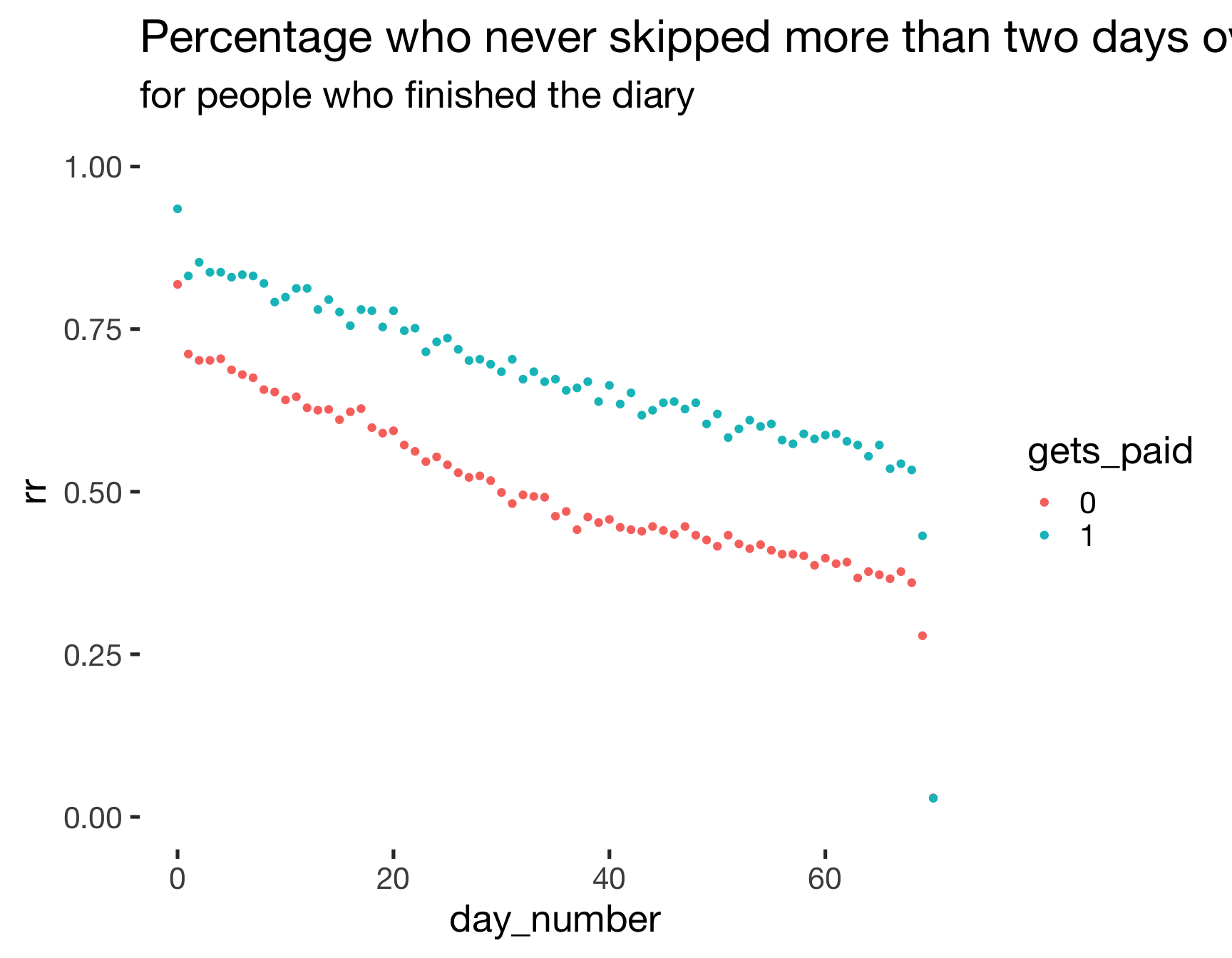mean_finishing_personality_by_payment <- s1_demo %>%
left_join(s1_filter, by = 'session', suffix = c("_demo", "")) %>%
left_join(s2_initial,by ='session', suffix = c("", "_initial")) %>%
group_by(gets_paid) %>%
summarise(finish_demo = n_nonmissing(ended_demo),
finish_initial = n_nonmissing(ended_initial), n = n(),
prc_ini_over_demo = round(100*finish_initial/finish_demo),
consc = mean(bfi_consc,na.rm=T))
kable(mean_finishing_personality_by_payment)
gets_paid finish_demo finish_initial n prc_ini_over_demo consc
0 1050 871 1050 83 3.577
1 557 540 557 97 3.478
NA 0 0 53 NaN NaN
mean_finishing_follow_up_by_payment <- s1_filter %>% select(-ended) %>% right_join(s2_initial,by ='session') %>% left_join(s4_followup, by = 'session', suffix = c("_initial", "_followup")) %>% group_by(gets_paid) %>% summarise(followup = n_nonmissing(ended_followup), initial = n_nonmissing(ended_initial), n(), prc_fu_over_initial = round(100*followup/initial))
kable(mean_finishing_follow_up_by_payment)
gets_paid followup initial n() prc_fu_over_initial
0 656 871 958 75
1 484 540 554 90
fertile_known_man_known = diary_social %>% group_by(session, created) %>%
summarise(n = n_nonmissing(person_attractiveness_short_term)) %>%
group_by(session) %>% summarise(n = sum(n)) # fertility known, man known
fertile_known_man_known$n %>% qplot() + xlim(1,NA)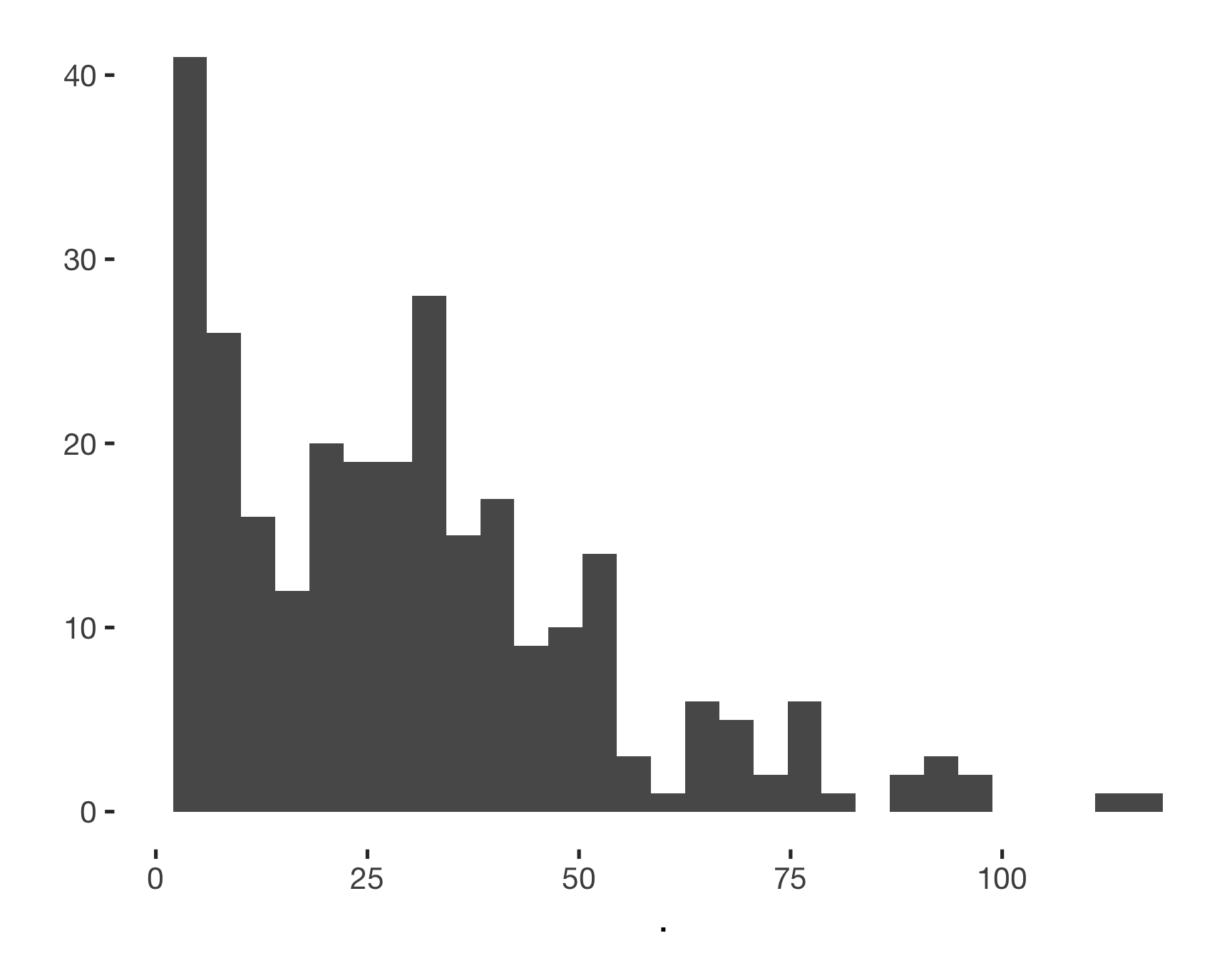fertile_known_person_known = diary_social %>% group_by(session, created) %>% summarise(n = n_nonmissing(person_sex)) %>% group_by(session) %>% summarise(n = sum(n)) # fertility known, man known finished_timespent = s4_timespent %>% group_by(session) %>% summarise(n = n_nonmissing(ended)) %>% filter(n >= 1) %>% nrow() timespent_gt3 = s4_timespent %>% group_by(session) %>% summarise(n = n_nonmissing(ended)) %>% filter(n > 3) %>% nrow() timespent_men = network %>% group_by(session) %>% filter(person_sex == 2, person_relationship_to_anchor != "biological_relative") %>% summarise(n = n_nonmissing(ended)) %>% filter(n > 0) %>% nrow() timespent_3men = network %>% group_by(session) %>% filter(person_sex == 2, person_relationship_to_anchor != "biological_relative") %>% summarise(n = n_nonmissing(ended)) %>% filter(n > 2) %>% nrow() fertile_known_man_known_participants = fertile_known_man_known %>% filter(n > 0) %>% nrow() fertile_known_person_known_participants = fertile_known_person_known %>% filter(n > 0) %>% nrow() finished_followup = sum(!is.na(s4_followup$ended))

# all_surveys %>% mutate(
#   should_be_done = created_demo + days(70) < today()
# ) %>% filter(should_be_done == T) %>% xtabs(~ is.na(ended), .)

# all_surveys %>% mutate(
# should_be_done = created_demo + days(70) < today()
# ) %>% left_join(s3_daily %>% group_by(session) %>% summarise(n = n_nonmissing(ended))) %>%
#  filter(should_be_done == T, n > 40) %>% xtabs(~ is.na(ended), .)

all_surveys %>% mutate(should_be_done = created_demo + days(70) < today()) %>%
left_join( s4_timespent %>% group_by(session) %>% summarise(n_timespent = n_nonmissing(ended)), by = 'session') %>%
filter(hetero_relationship == 0, should_be_done == T, n_timespent > 0, !is.na(n_timespent)) %>% nrow()
##  349
all_surveys %>% mutate(should_be_done = created_demo + days(70) < today()) %>%
left_join( s4_timespent %>% group_by(session) %>% summarise(n_timespent =  n_nonmissing(ended)), by = 'session') %>%
filter(hetero_relationship == 0 & should_be_done == T & (is.na(n_timespent) | n_timespent == 0)) %>% nrow()
##  184
1. 1660 signed up for the study.
2. 1607 finished the demographics (533 singles, 1091 in relationship).
3. 1373 finished the demographic and personality questionnaires.
4. 1345 submitted at least one diary entry (>= 20: 1075, >= 50 774). On average participants completed 44.5645 diary entries.
5. 349 singles reported information on least one person mentioned during the diary (>3: 293, at least one man who wasn’t biologically related: 282, >2men: 124).
6. 1140 (83% of those who finished the intake survey) finished the follow-up survey. Finishers completed on average 50.316 diary entries.

# Contraception

library(UpSetR)
comma_separated_to_columns <- function(df, col) {
colname <- deparse(substitute(col))
df$splitcol <- df %>% pull(colname) separate_rows(df, splitcol, convert = TRUE, sep = ", ") %>% mutate(splitcol = if_else(is.na(splitcol), "no", if_else(splitcol == "" | splitcol %in% c(), "other", as.character(splitcol)))) %>% mutate(#splitcol = stringr::str_c(colname, "_", splitcol), value = 1) %>% spread(splitcol, value, fill = 0) %>% select(-colname) } library(UpSetR) all_surveys %>% select(session, contraception_method) %>% comma_separated_to_columns(contraception_method) %>% select(-session) %>% as.data.frame() %>% { upset(., ncol(.), 20, show.numbers = TRUE, order.by = "freq", main.bar.color = "#6E8691", matrix.color = "#6E8691", sets.bar.color = "#53AC9B") }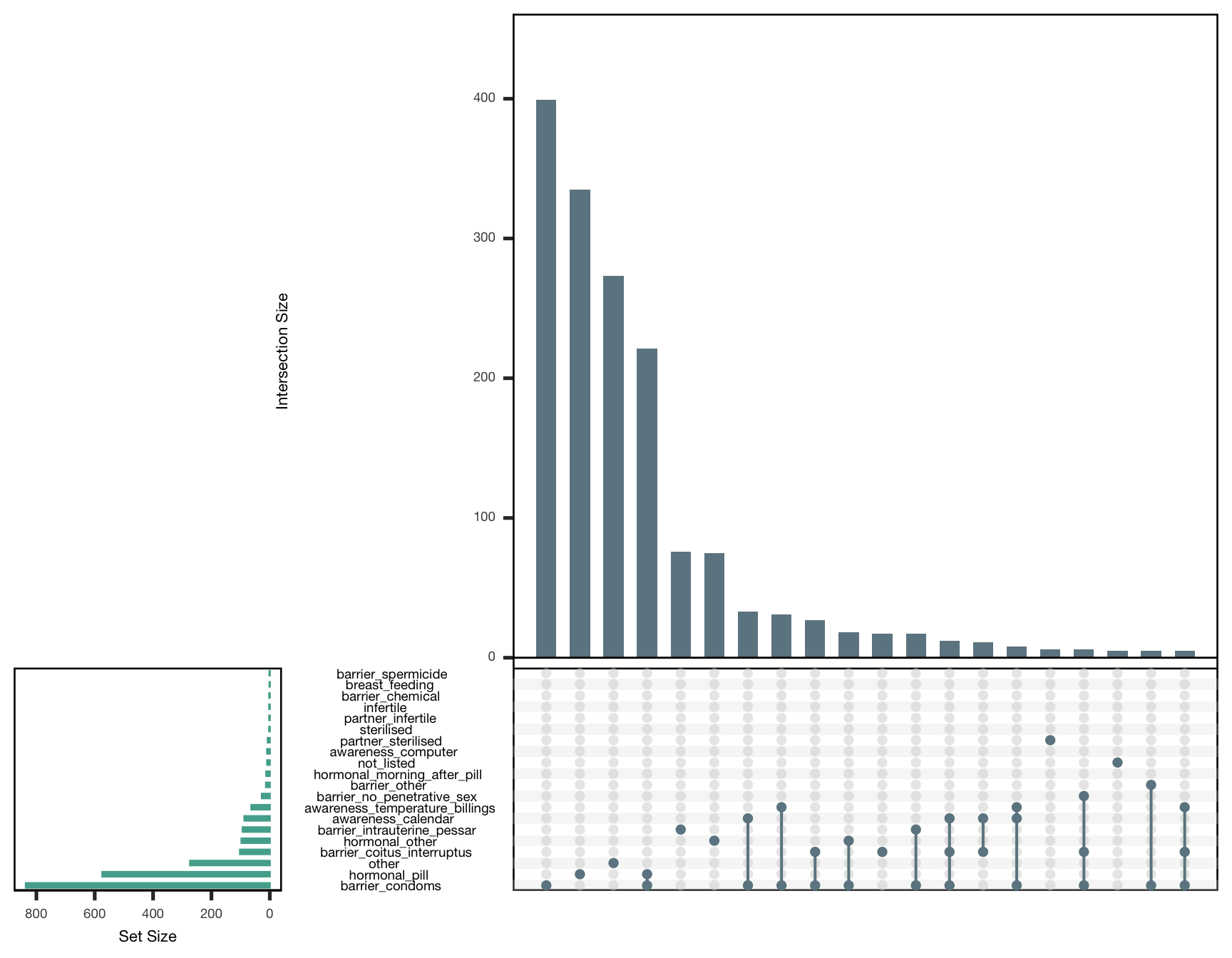## social network summary(as.factor(network$person_sex))
##    1    2    3 NA's
## 1160  796  160  129
by_sex = s4_timespent %>%
group_by(session) %>%
summarise(female = sum(person_sex == 1, na.rm=T), male = sum(person_sex == 2, na.rm=T), n= n()) %>% select(female, male,n)
total_network = nrow(network)
persons= nrow(network %>% filter(person_remember == 1 & !is.na(ended)))

2245 number of persons who were mentioned in diary 2118 number of nicknames/names women remembered.

## Comparison with quasi-control group

### Comparisons on key variables

women <- all_surveys %>% filter(reasons_for_exclusion == "")
dig1 <- function(x) { sprintf("%.1f", x) }
dig2 <- function(x) { sprintf("%.2f", x) }
pct <- function(x) { sprintf("%.0f", x*100) }

remove_p_values <- function(planned_plot, call_parts = 3:10) {
subtitle <- as.list(planned_plot$labels$subtitle)
subtitle[call_parts] <- NULL
planned_plot$labels$subtitle <- as.call(subtitle)
planned_plot
}
comps = data_frame(Variable = character(0),
HC user - Mean (SD) = character(0),
Cycling - Mean (SD) = character(0),
99% CI = character(0),
)

compare_by_group = function(var, data, type = "np", ...) {
var_s <- enquo(var)
var <- rlang::as_name(var_s)
sd_hc = sd(data[data$hormonal_contraception == 1,][[var]], na.rm = T) sd_nc = sd(data[data$hormonal_contraception == 0,][[var]], na.rm = T)
data$Hormonal contraception = forcats::fct_relevel( forcats::fct_recode(forcats::as_factor(data$hormonal_contraception), "Cycling" = "FALSE", "HC user" = "TRUE"), "HC user")
comp = as.formula(paste0("",var, " ~ Hormonal contraception"))
tt = t.test(comp, data = data, conf.level = 0.99)

summary = data_frame(Variable = var,
HC user - Mean (SD) = paste0(dig1(tt$estimate)," (", dig1(sd_hc),")"), Cycling - Mean (SD) = paste0(dig1(tt$estimate), " (", dig1(sd_nc), ")"),
99% CI = paste(dig1(tt$conf.int), collapse = ";") ) rownames(summary) = NULL comps <<- bind_rows(comps, summary) data[[var]] <- data[[var]] %>% zap_attributes() planned_plot <- ggstatsplot::ggbetweenstats( data = data, x = Hormonal contraception, y = !! var_s, messages = FALSE, conf.level = 0.99, type = type, ..., ggtheme = theme_cowplot(font_size = 18) ) + # further modification outside of ggstatsplot scale_color_manual("", values = c("Cycling" = "red", "HC user" = "black"), guide = F) + # scale_x_discrete("Hormonal contraception", labels = c("1" = "HC user", "2" = "Cycling")) + scale_fill_manual("", values = c("Cycling" = "red", "HC user" = "black"), guide = F) remove_p_values(planned_plot) } library(ggstatsplot) library(cowplot) women$Age (years) <- women$age compare_by_group(Age (years), women)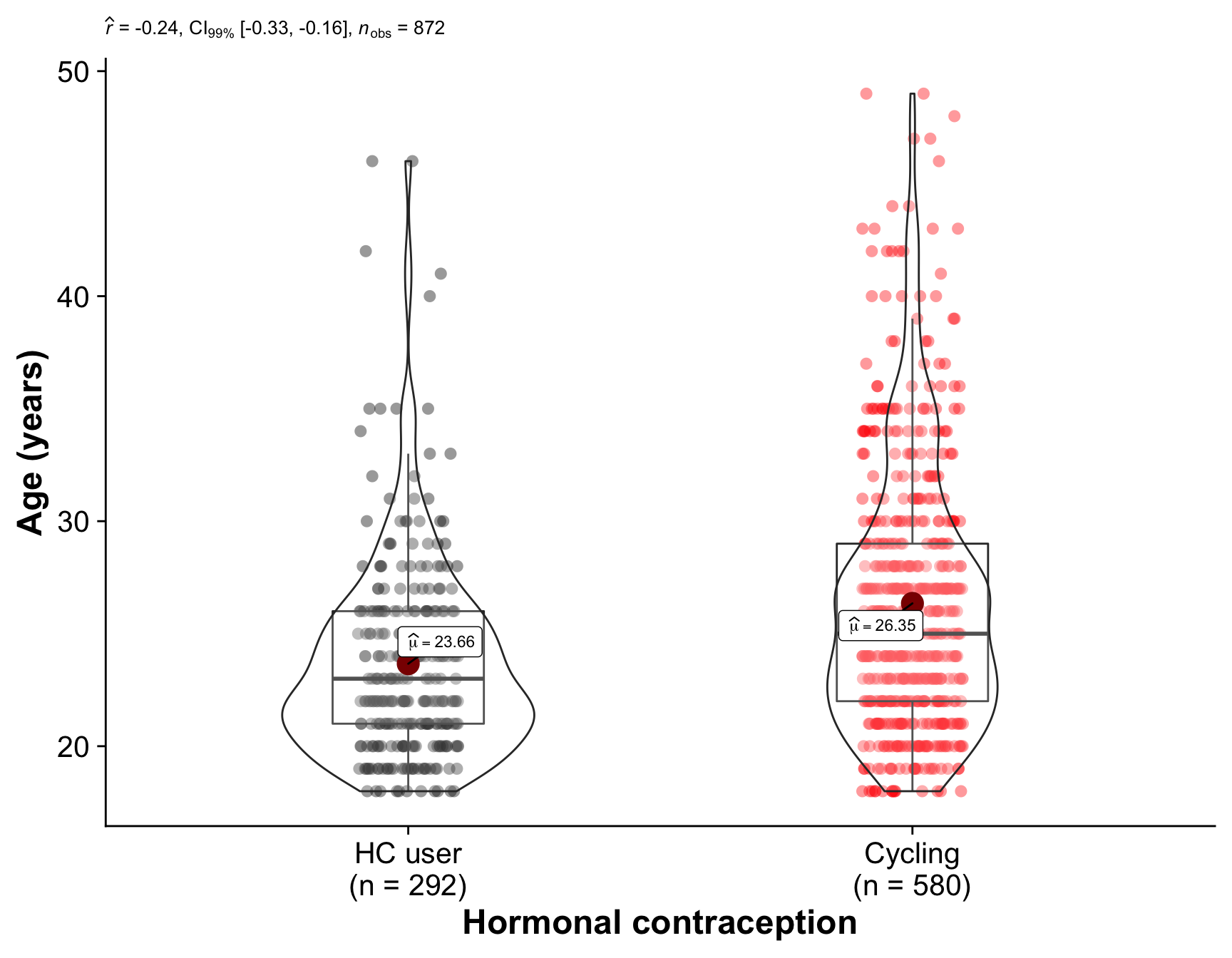Comparison of the hormonal contraception users (our quasi-control group) with women who are regularly cycling. The plot shows the distribution of values in each group and the results of a Mann-Whitney U test. { ggbarstats(women %>% mutate(relationship_status=as_factor(relationship_status)), relationship_status, hormonal_contraception, messages = FALSE, conf.level = 0.99, bf.message = F, ggtheme = theme_cowplot(font_size = 18) ) + # further modification outside of ggstatsplot scale_x_discrete("Hormonal contraception", labels = c("TRUE" = "HC user", "FALSE" = "Cycling")) } %>% remove_p_values(call_parts = 3:12)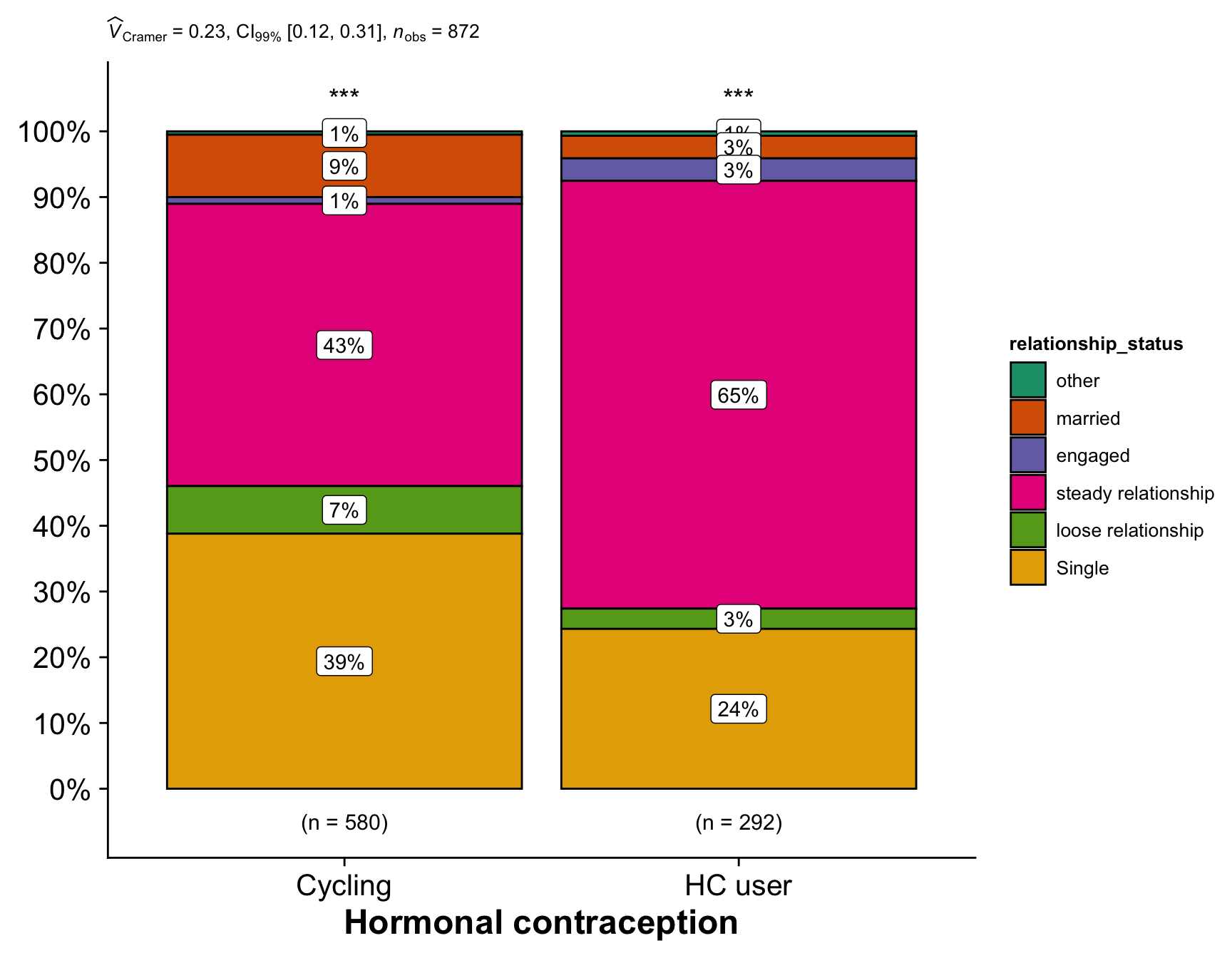Comparison of the hormonal contraception users (our quasi-control group) with women who are regularly cycling. The plot shows the distribution of categories in each group and the results of a Chi-Square test for equal distribution. women$Age at first sex (years) <- women$first_time compare_by_group(Age at first sex (years), women)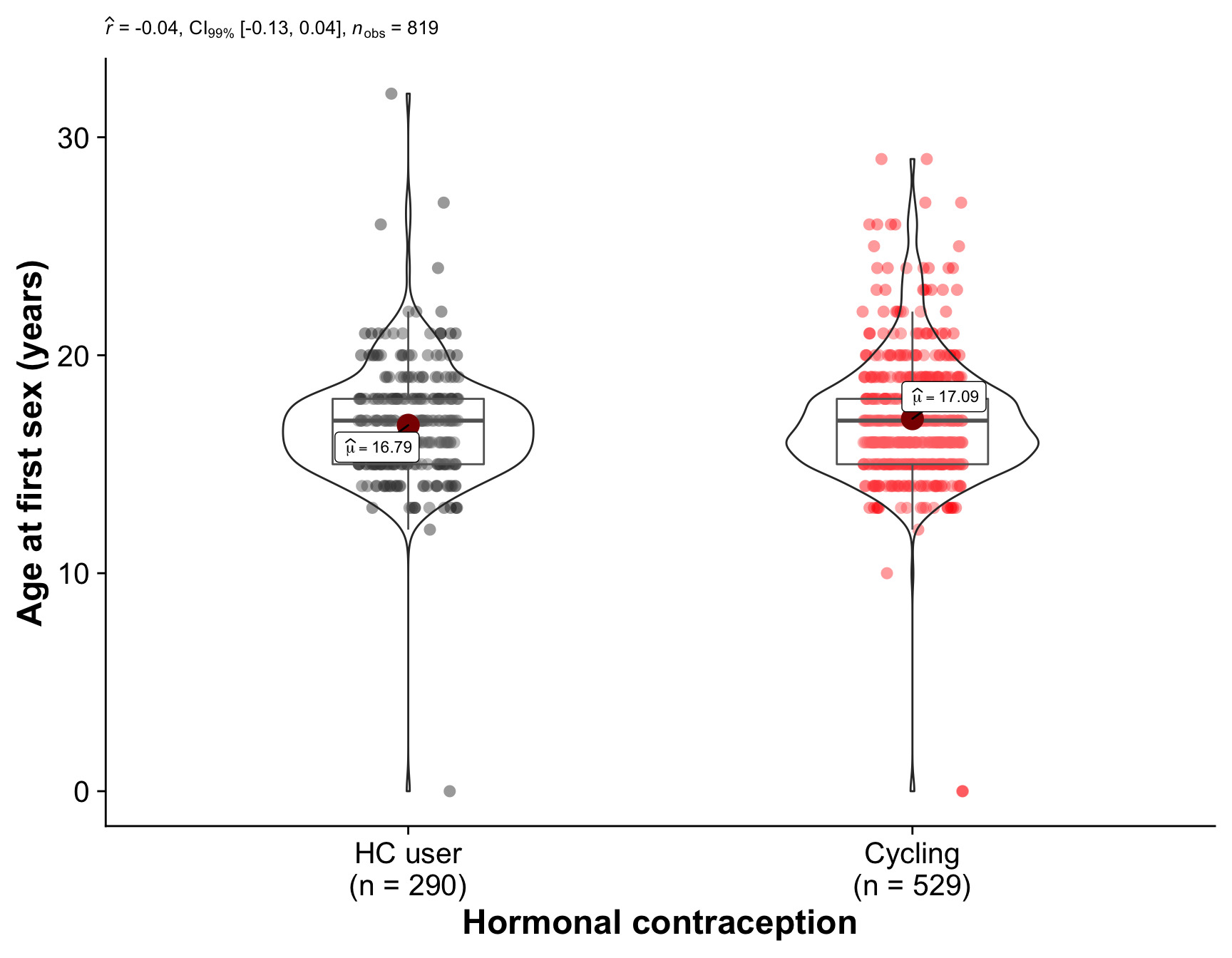Comparison of the hormonal contraception users (our quasi-control group) with women who are regularly cycling. The plot shows the distribution of values in each group and the results of a Mann-Whitney U test. women$Age at menarche (years) <- women$menarche compare_by_group(Age at menarche (years), women)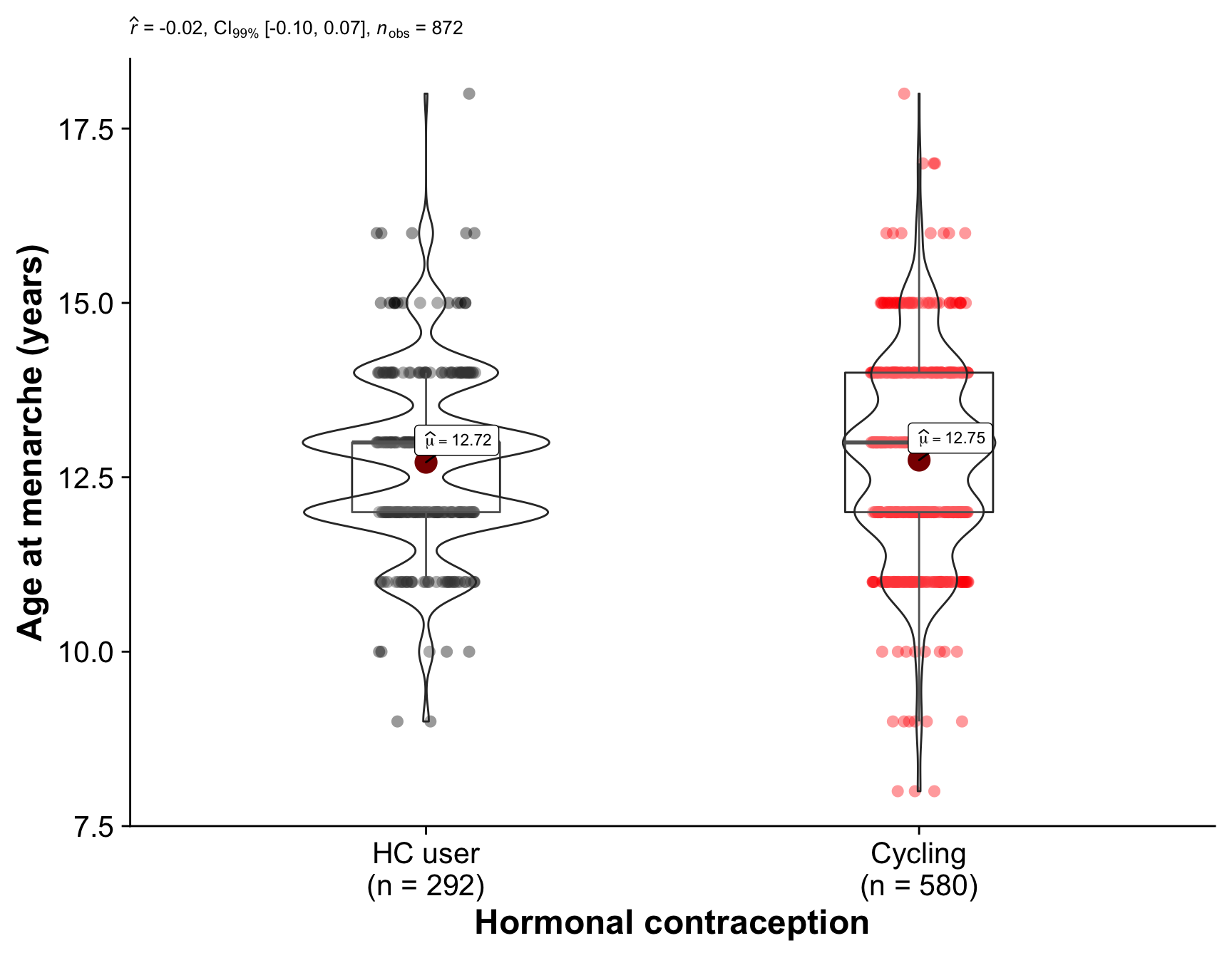Comparison of the hormonal contraception users (our quasi-control group) with women who are regularly cycling. The plot shows the distribution of values in each group and the results of a Mann-Whitney U test. women$Relationship duration (years) <- women$relationship_duration compare_by_group(Relationship duration (years), women) + scale_y_sqrt(breaks = c(1,5, 10, 20, 30))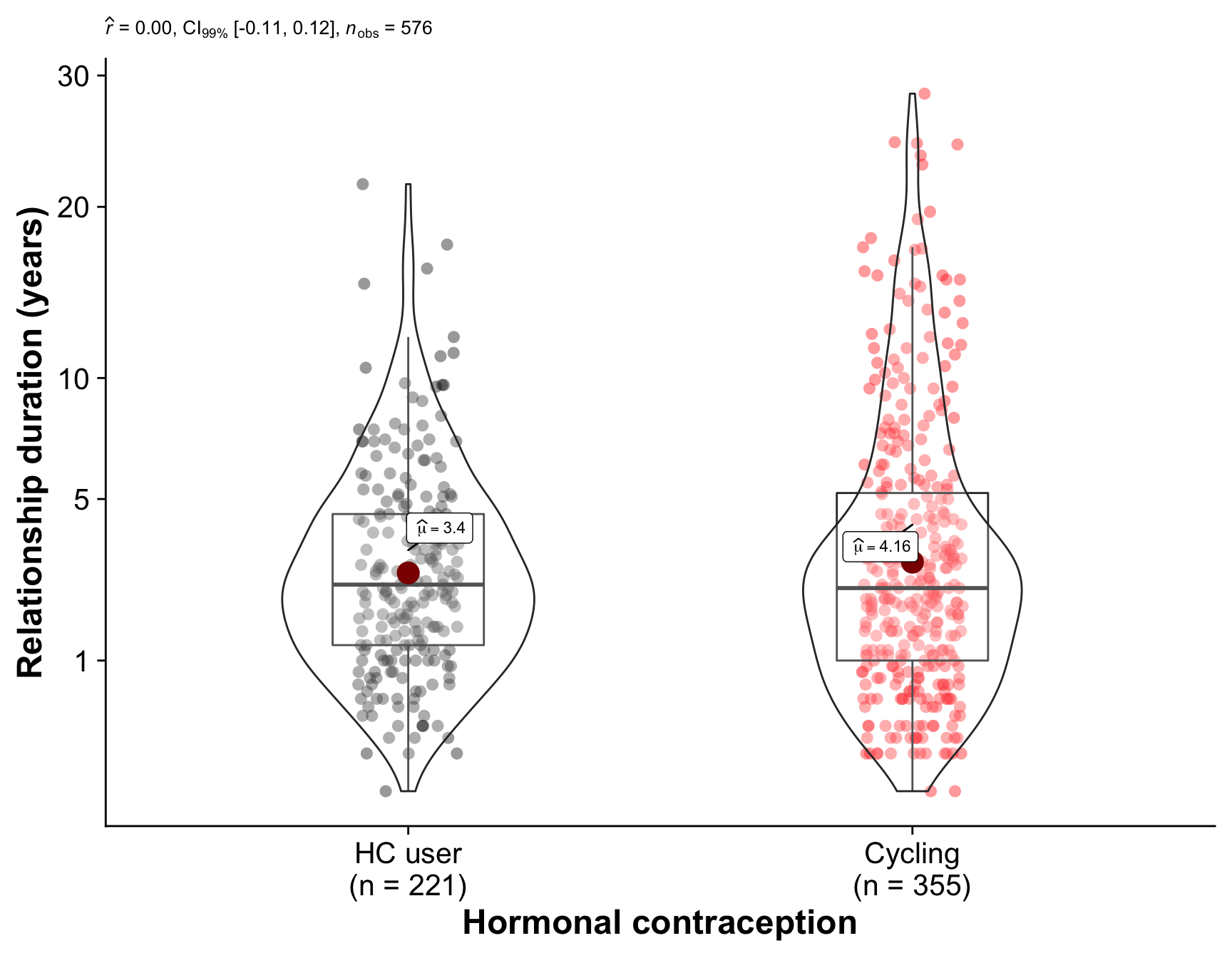Comparison of the hormonal contraception users (our quasi-control group) with women who are regularly cycling. The plot shows the distribution of values in each group and the results of a Mann-Whitney U test. women$Avg. cycle length (days) <- women$menstruation_length compare_by_group(Avg. cycle length (days), women)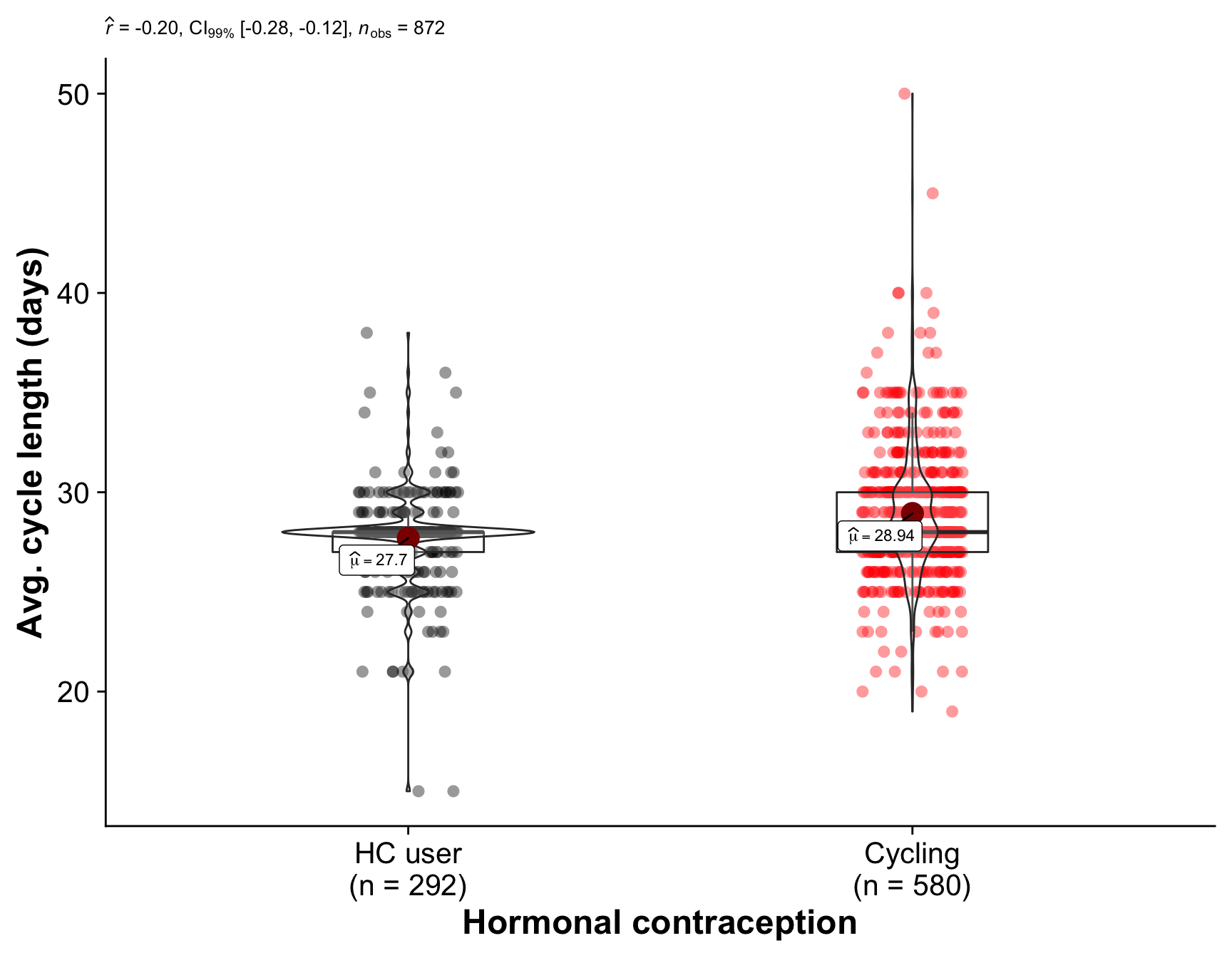Comparison of the hormonal contraception users (our quasi-control group) with women who are regularly cycling. The plot shows the distribution of values in each group and the results of a Mann-Whitney U test. women$Cycle regularity <- women$menstruation_regularity compare_by_group(Cycle regularity, women) + scale_y_continuous( labels = c("1" = "no fluctuation", "2" = "1-2 days", "3" = "3-5 days", "4" = ">5 days"))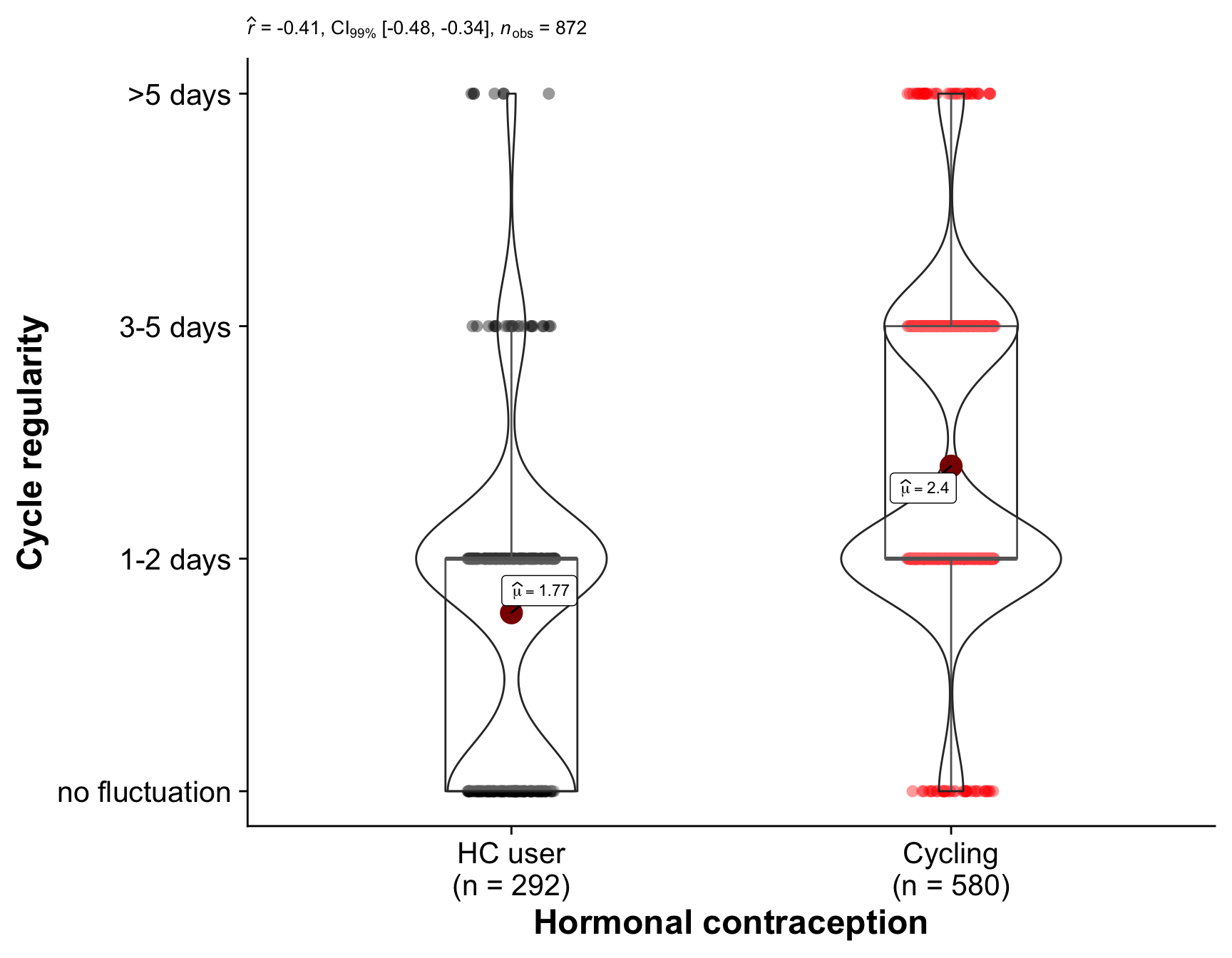Comparison of the hormonal contraception users (our quasi-control group) with women who are regularly cycling. The plot shows the distribution of values in each group and the results of a Mann-Whitney U test. women$No. lifetime sexual partners <- women$number_sexual_partner compare_by_group(No. lifetime sexual partners, women, type = "r") %>% remove_p_values(call_parts = 3:4) + scale_y_sqrt(breaks = c(1,5, 10, 20, 50, 100))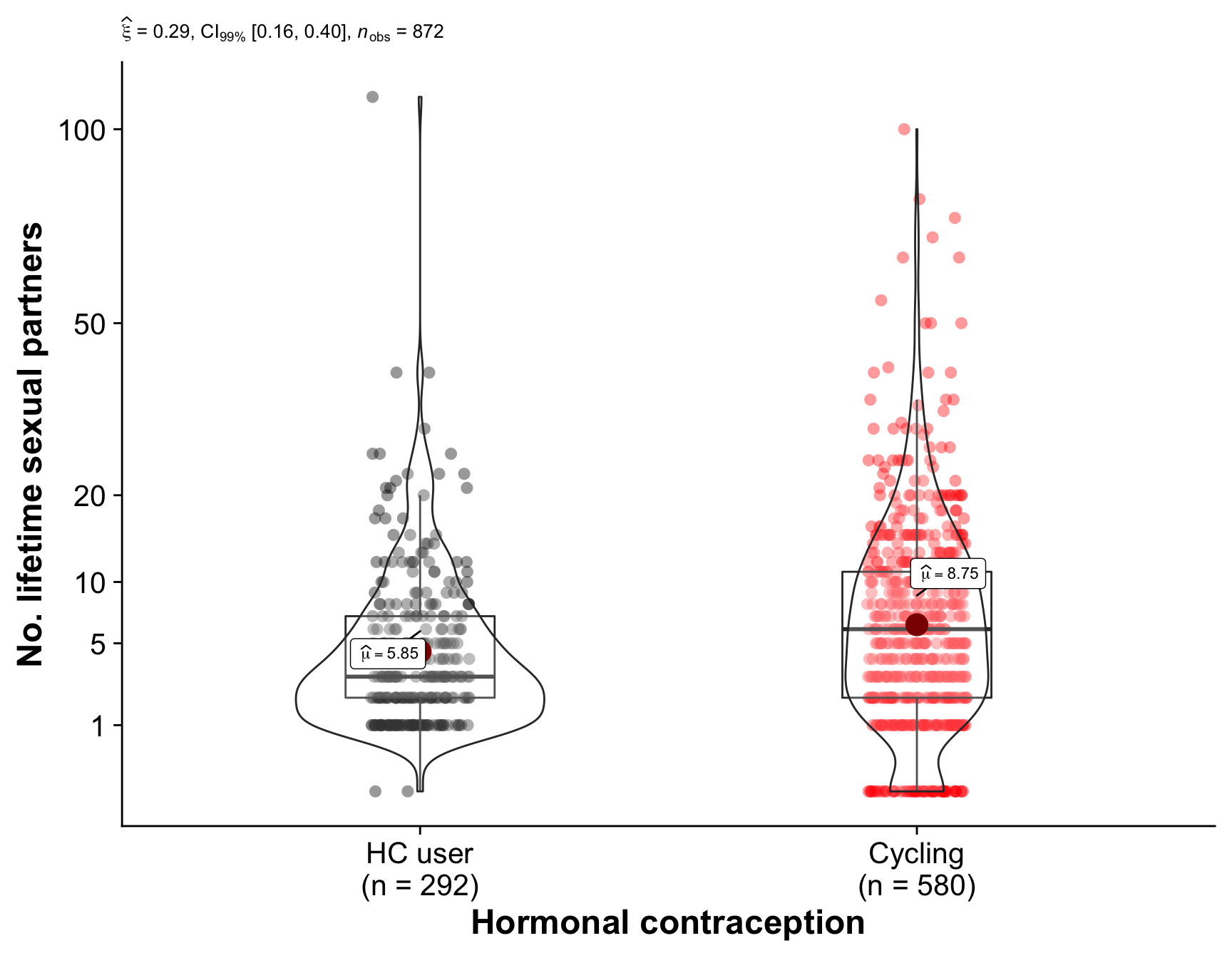Comparison of the hormonal contraception users (our quasi-control group) with women who are regularly cycling. The plot shows the distribution of values in each group and the results of Yuen’s test for trimmed means (10% trimmed). women$BFI Extraversion <- women$bfi_extra compare_by_group(BFI Extraversion, women)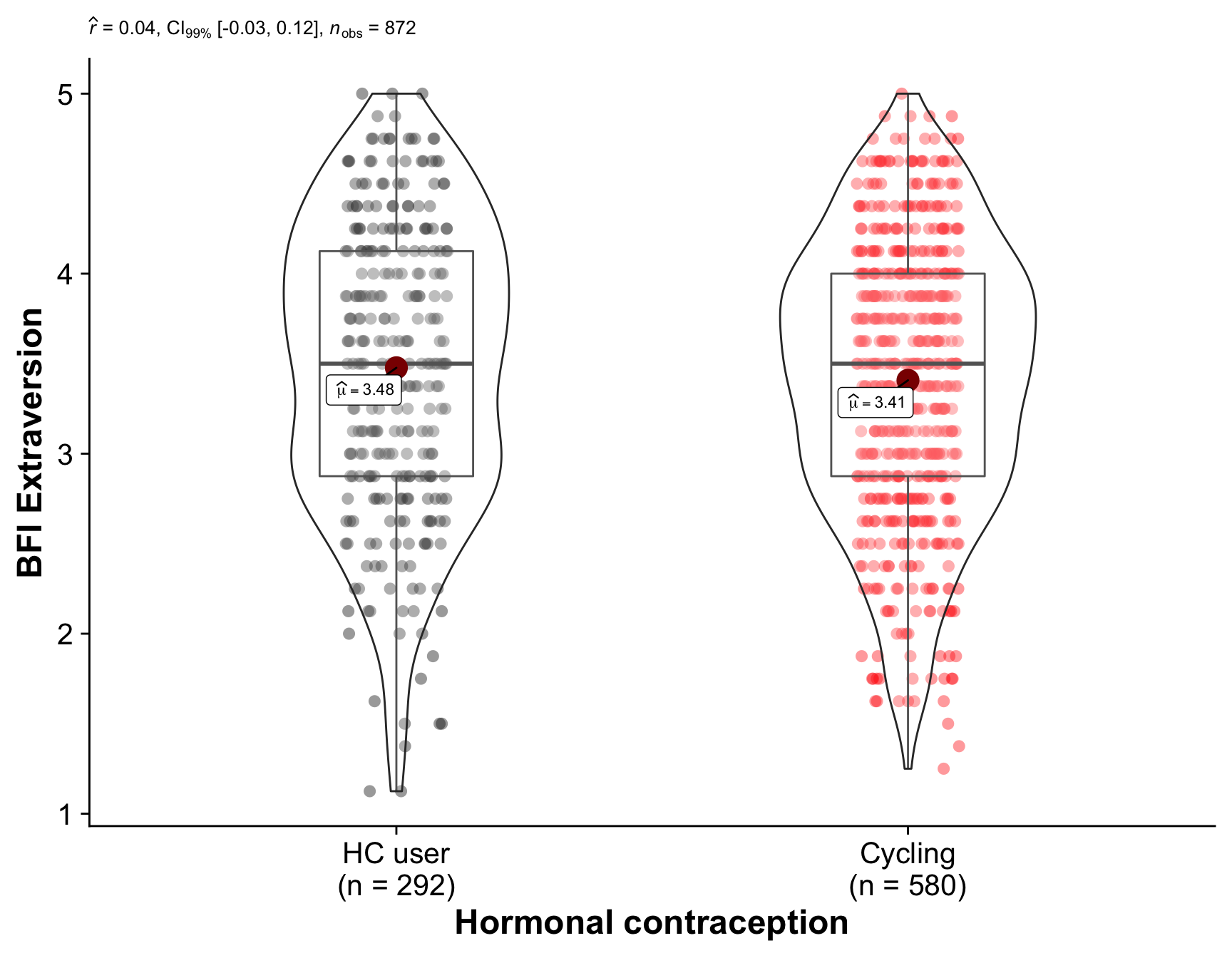Comparison of the hormonal contraception users (our quasi-control group) with women who are regularly cycling. The plot shows the distribution of values in each group and the results of a Mann-Whitney U test. women$BFI Agreeableness <- women$bfi_agree compare_by_group(BFI Agreeableness, women)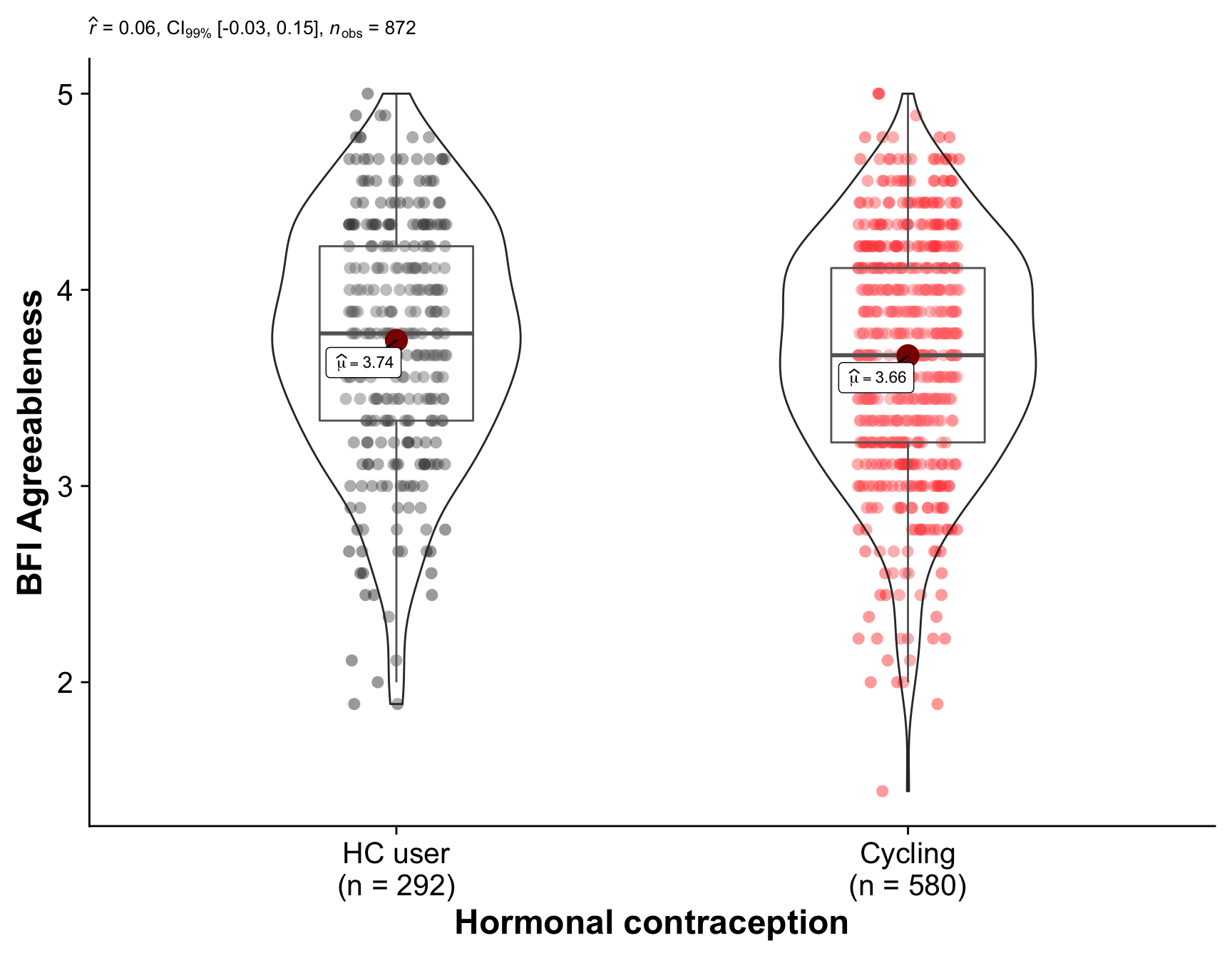Comparison of the hormonal contraception users (our quasi-control group) with women who are regularly cycling. The plot shows the distribution of values in each group and the results of a Mann-Whitney U test. women$BFI Neuroticism <- women$bfi_neuro compare_by_group(BFI Neuroticism, women)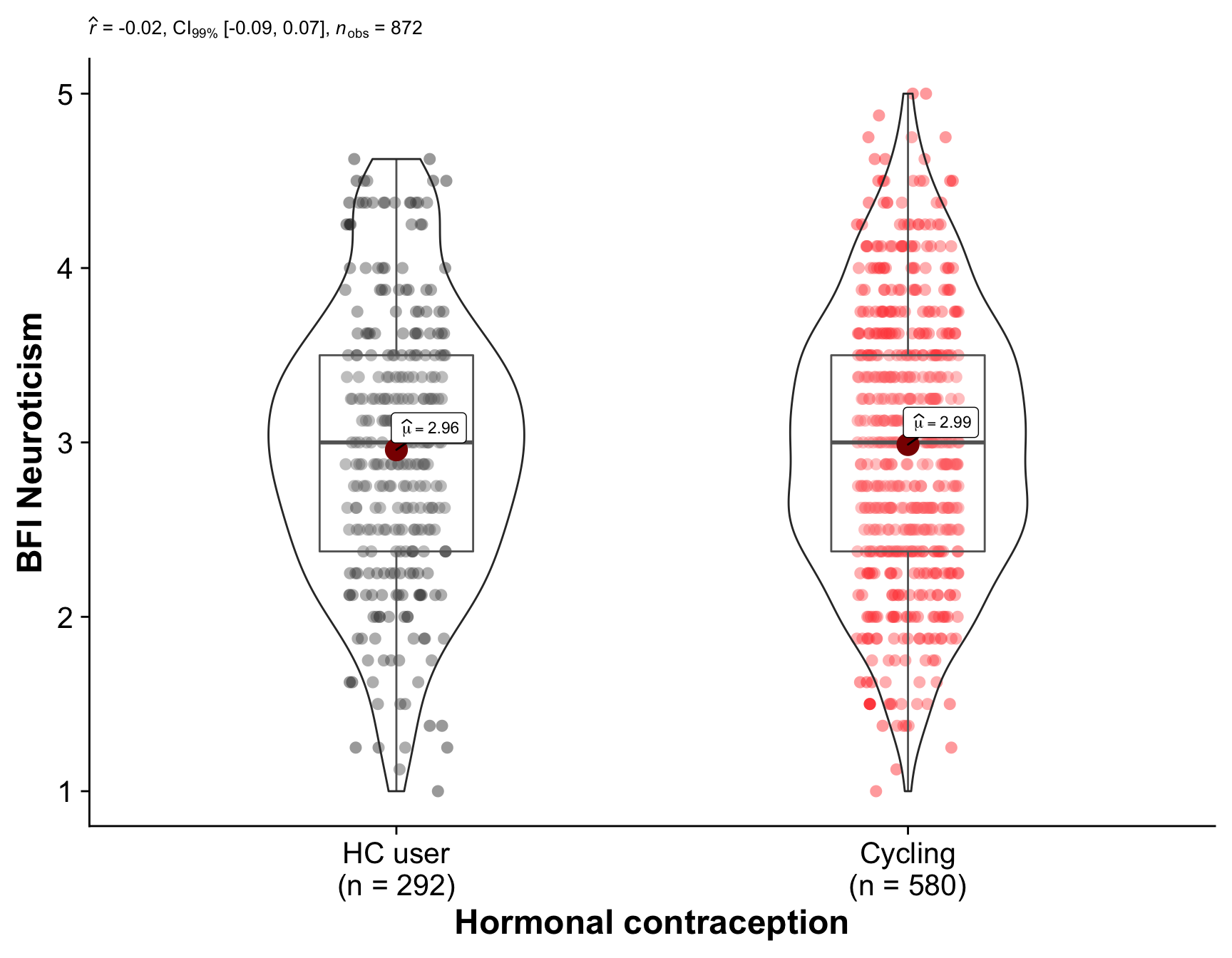Comparison of the hormonal contraception users (our quasi-control group) with women who are regularly cycling. The plot shows the distribution of values in each group and the results of a Mann-Whitney U test. women$BFI Conscientiousness <- women$bfi_consc compare_by_group(BFI Conscientiousness, women)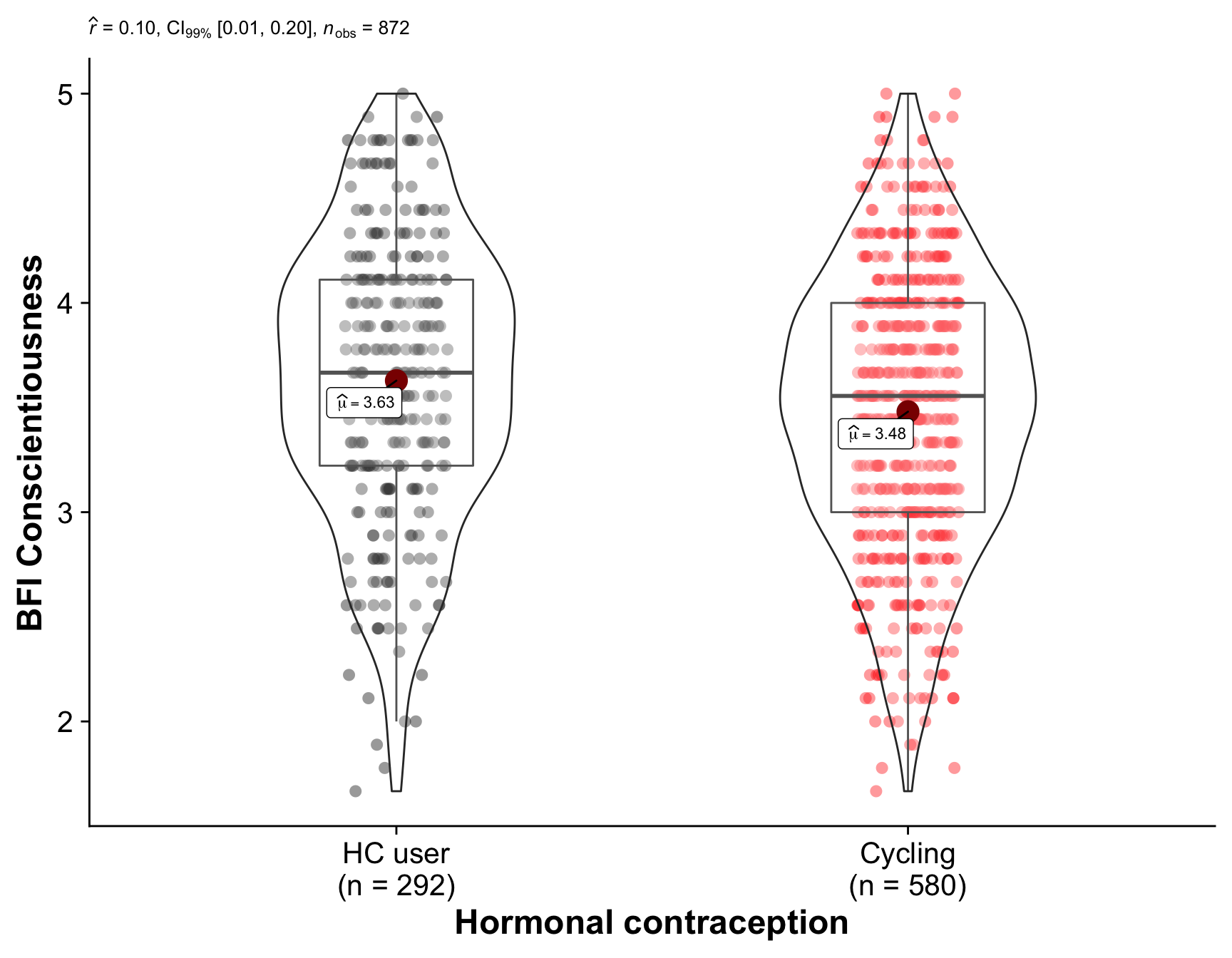Comparison of the hormonal contraception users (our quasi-control group) with women who are regularly cycling. The plot shows the distribution of values in each group and the results of a Mann-Whitney U test. women$BFI Openness <- women$bfi_open compare_by_group(BFI Openness, women)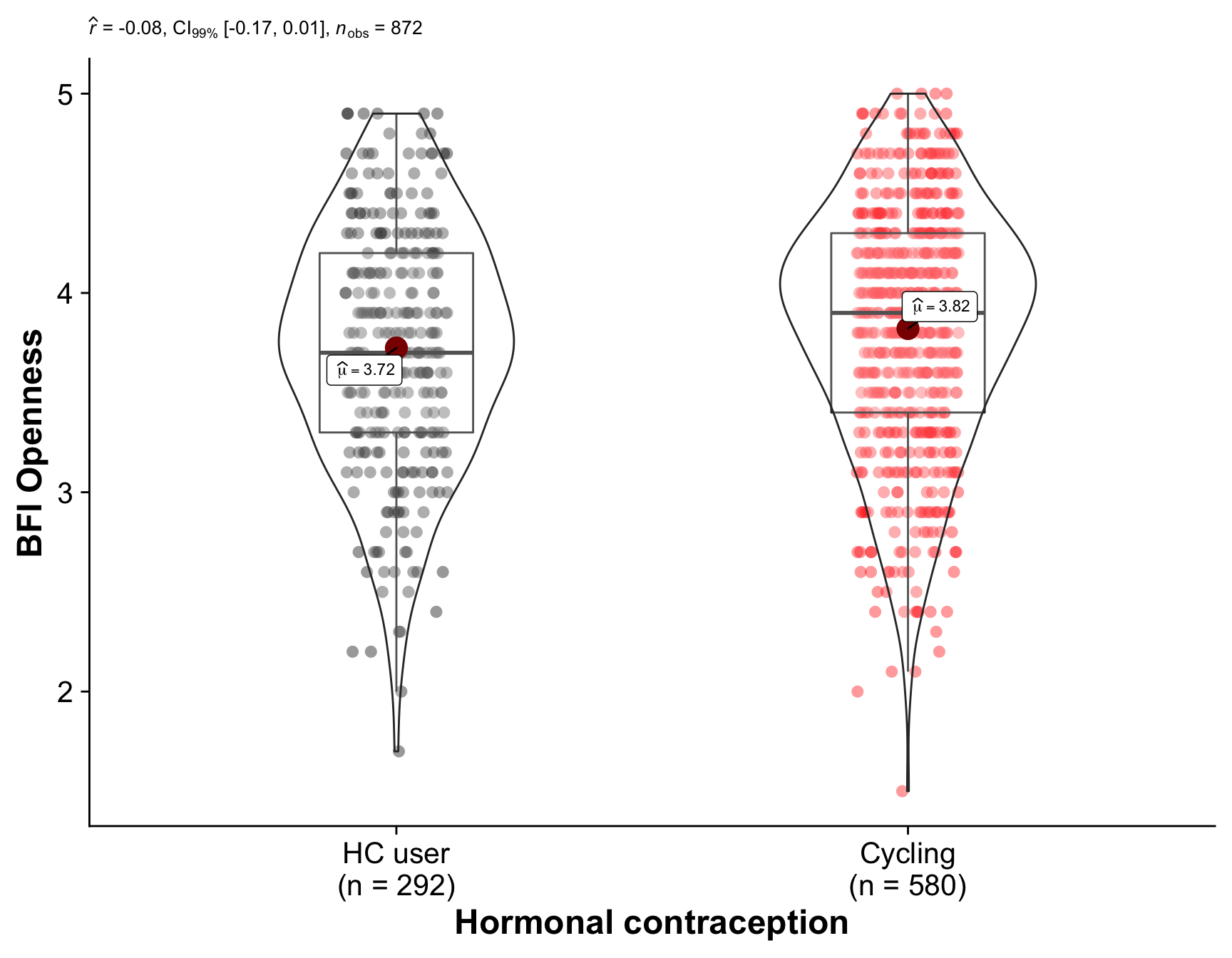Comparison of the hormonal contraception users (our quasi-control group) with women who are regularly cycling. The plot shows the distribution of values in each group and the results of a Mann-Whitney U test. women$Sociosexuality Inventory Revised <- women$soi_r compare_by_group(Sociosexuality Inventory Revised, women)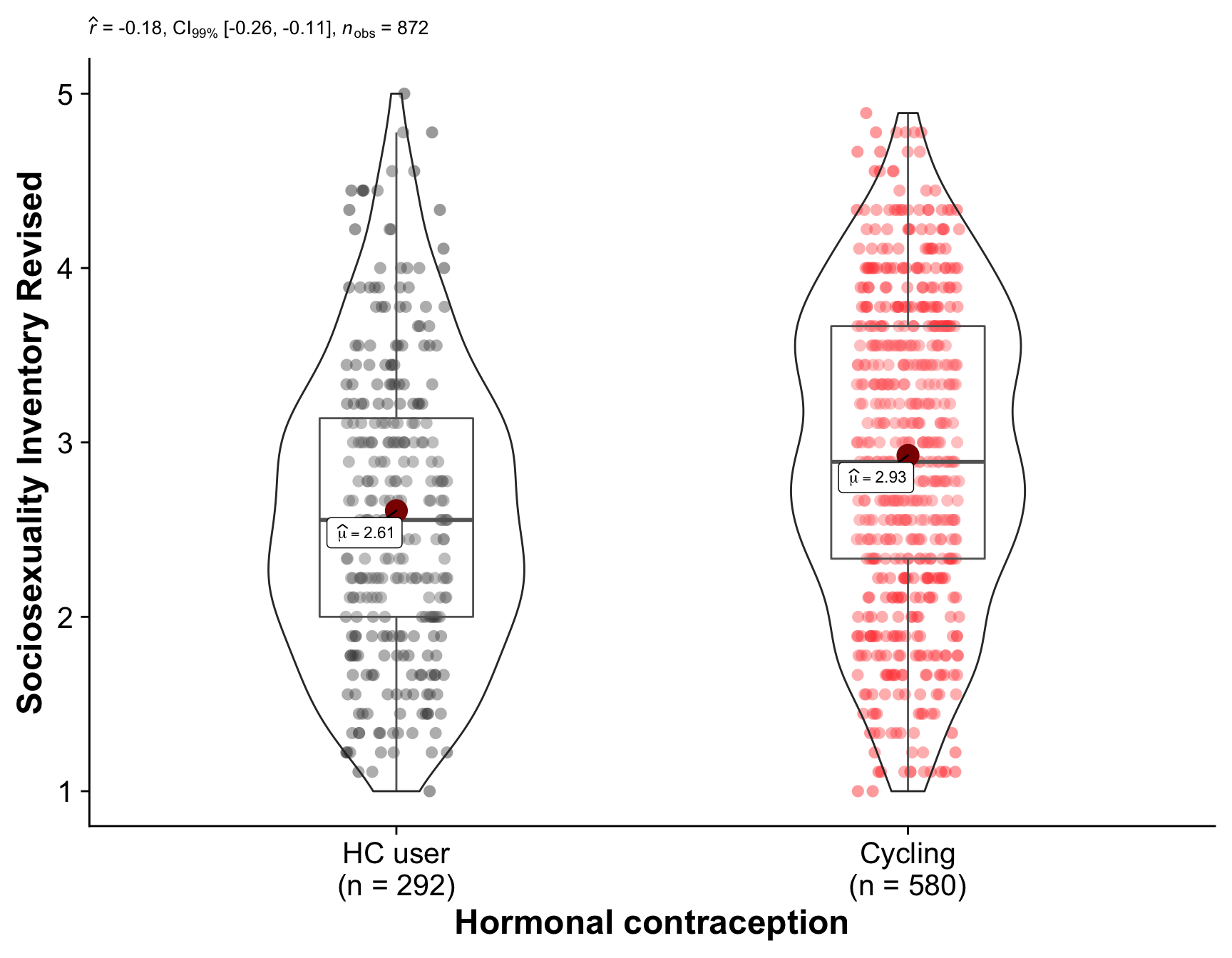Comparison of the hormonal contraception users (our quasi-control group) with women who are regularly cycling. The plot shows the distribution of values in each group and the results of a Mann-Whitney U test. women$Relationship satisfaction <- women\$relationship_satisfaction
compare_by_group(Relationship satisfaction, women)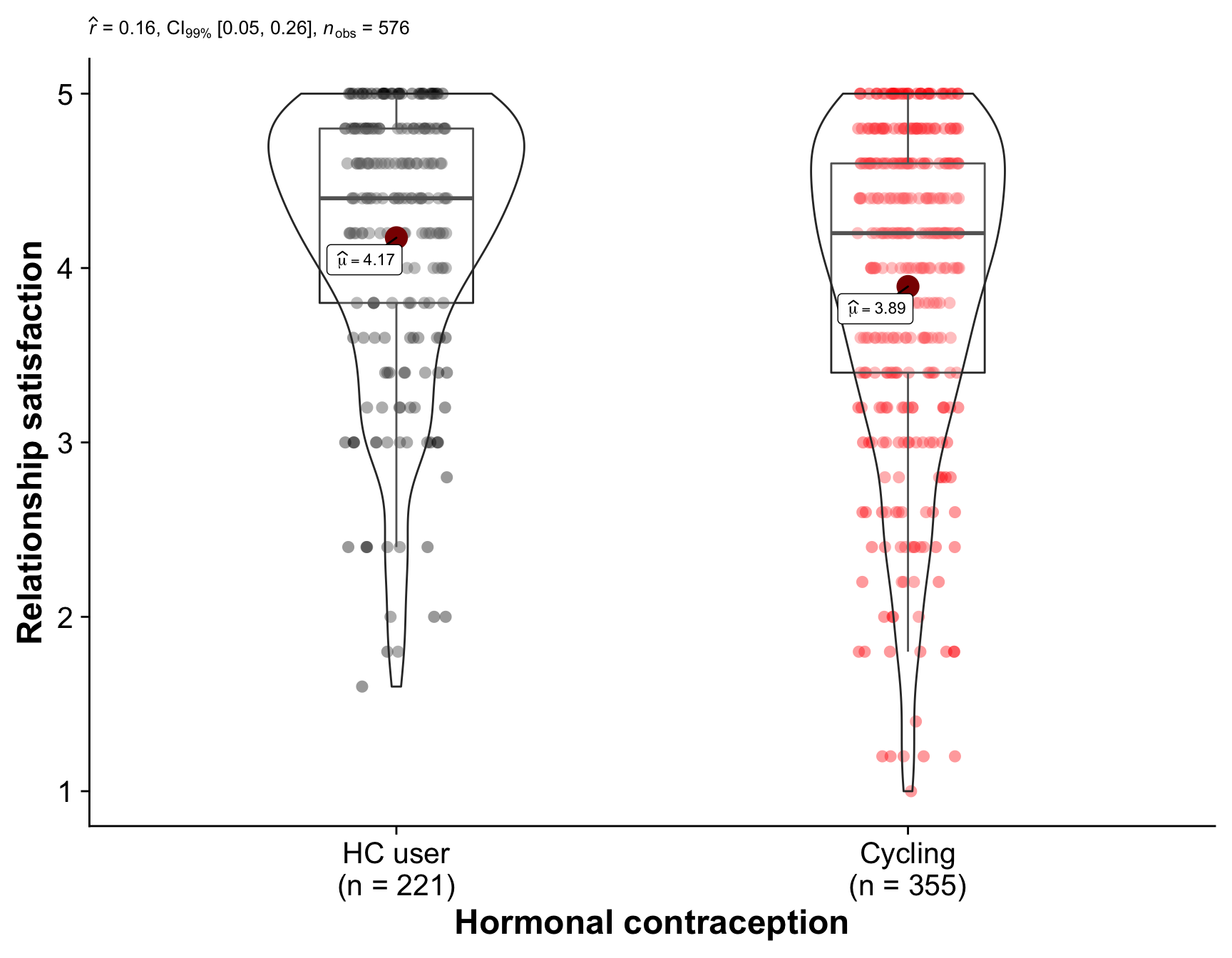Comparison of the hormonal contraception users (our quasi-control group) with women who are regularly cycling. The plot shows the distribution of values in each group and the results of a Mann-Whitney U test.

compare_by_group(religiosity, women)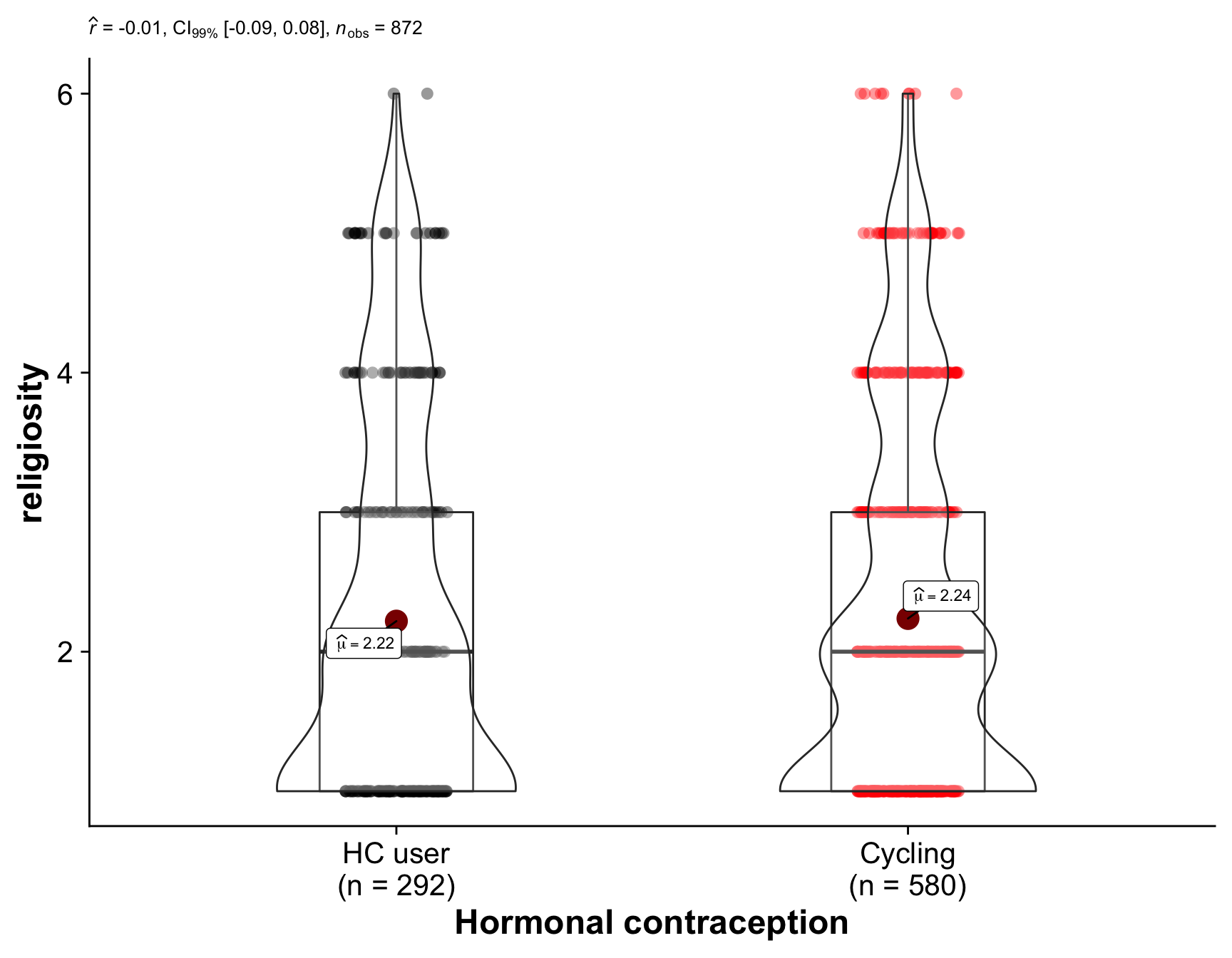Comparison of the hormonal contraception users (our quasi-control group) with women who are regularly cycling. The plot shows the distribution of values in each group and the results of a Mann-Whitney U test.

{ ggbarstats(women,
net_income,
hormonal_contraception,
messages = FALSE,
bf.message = F,
conf.level = 0.99,
ggtheme = theme_cowplot(font_size = 18)
) + # further modification outside of ggstatsplot
scale_x_discrete("Hormonal contraception", labels = c("TRUE" = "HC user", "FALSE" = "Cycling"))} %>%
remove_p_values(call_parts = 3:12)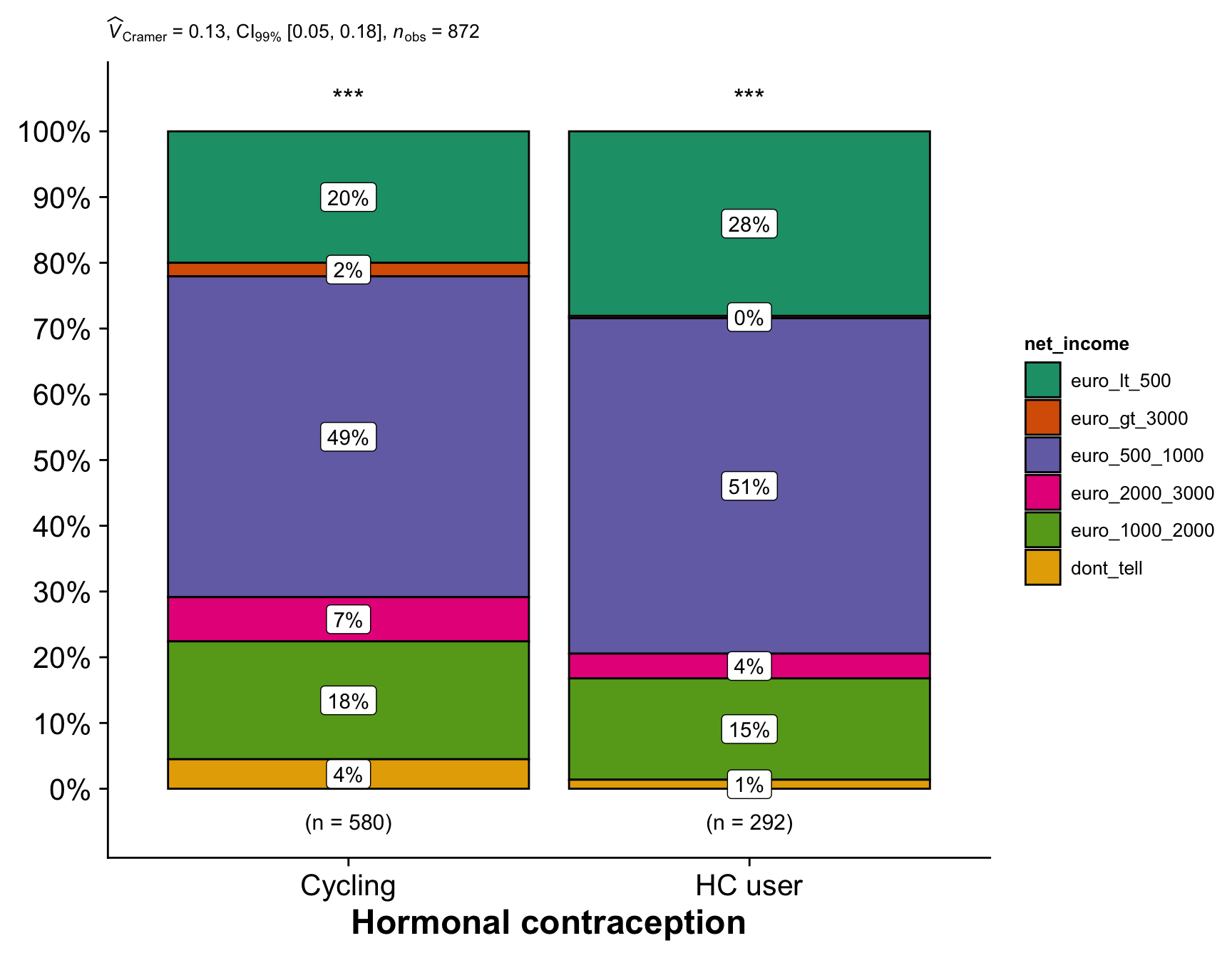Comparison of the hormonal contraception users (our quasi-control group) with women who are regularly cycling. The plot shows the distribution of categories in each group and the results of a Chi-Square test for equal distribution.

{ ggbarstats(women,
living_situation,
hormonal_contraception,
messages = FALSE,
conf.level = 0.99,
bf.message = F,
ggtheme = theme_cowplot(font_size = 18)
) + # further modification outside of ggstatsplot
scale_x_discrete("Hormonal contraception", labels = c("TRUE" = "HC user", "FALSE" = "Cycling")) } %>%
remove_p_values(call_parts = 3:12)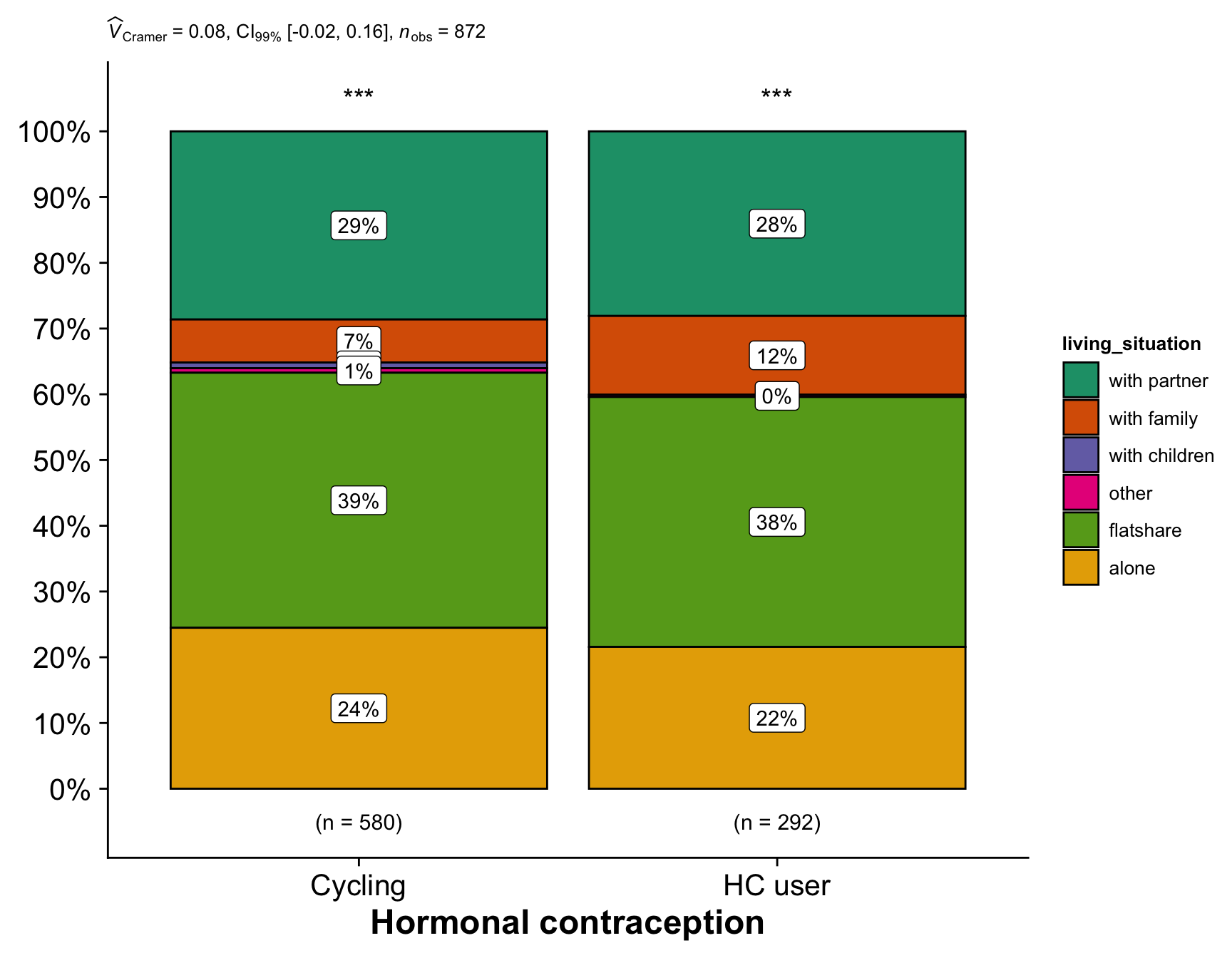Comparison of the hormonal contraception users (our quasi-control group) with women who are regularly cycling. The plot shows the distribution of categories in each group and the results of a Chi-Square test for equal distribution.

## Summary

comps %>%
kable(caption = "Means, SDs, and differences between both groups for all continuous variables") %>%
footnote("99% CI interval of the difference.") %>%
kable_styling()
Means, SDs, and differences between both groups for all continuous variables
Variable HC user - Mean (SD) Cycling - Mean (SD) 99% CI
Age (years) 23.7 (4.4) 26.3 (5.9) -3.6;-1.8
Age at first sex (years) 16.8 (2.6) 17.1 (2.9) -0.8;0.2
Age at menarche (years) 12.7 (1.3) 12.7 (1.4) -0.3;0.2
Relationship duration (years) 3.4 (3.2) 4.2 (4.9) -1.6;0.1
Avg. cycle length (days) 27.7 (2.3) 28.9 (3.1) -1.7;-0.7
Cycle regularity 1.8 (0.7) 2.4 (0.7) -0.8;-0.5
No. lifetime sexual partners 5.8 (8.6) 8.8 (10.9) -4.7;-1.2
BFI Extraversion 3.5 (0.8) 3.4 (0.8) -0.1;0.2
BFI Agreeableness 3.7 (0.6) 3.7 (0.6) -0.0;0.2
BFI Neuroticism 3.0 (0.8) 3.0 (0.8) -0.2;0.1
BFI Conscientiousness 3.6 (0.7) 3.5 (0.7) 0.0;0.3
BFI Openness 3.7 (0.6) 3.8 (0.6) -0.2;0.0
Sociosexuality Inventory Revised 2.6 (0.8) 2.9 (0.9) -0.5;-0.2
Relationship satisfaction 4.2 (0.8) 3.9 (0.9) 0.1;0.5
religiosity 2.2 (1.4) 2.2 (1.4) -0.3;0.2
Note:
99% CI interval of the difference.

## Multiple regression

altogether = glm(hormonal_contraception ~ age  + living_situation + religiosity + bfi_extra + bfi_neuro + bfi_agree + bfi_consc + bfi_open + soi_r + first_time + relationship_status + log1p(number_sexual_partner) + has_children + net_income, data = women %>% mutate(relationship_status=as_factor(relationship_status)), family = binomial("probit"))
ggcoefstats(altogether, package = "ggsci", palette = "default_igv", p.adjust.method = "BH",
conv.level = 0.99, stats.labels = FALSE, point.color = "black") +
ggrepel::geom_text_repel(aes(label = sprintf("%.2f [%.2f;%.2f]", estimate, conf.low, conf.high)), size = 3.3)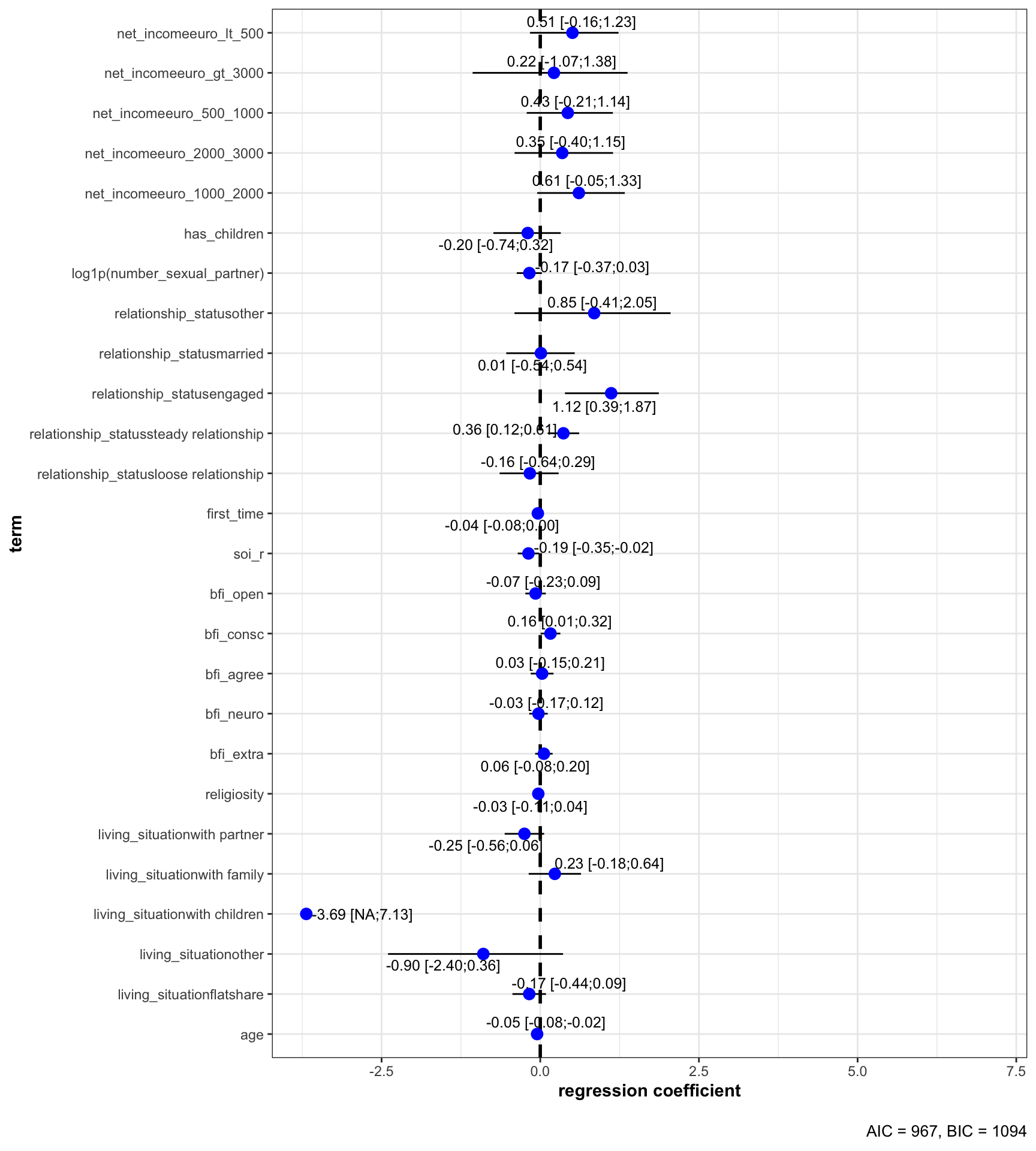A probit regression predicting hormonal contraception with many predictors at once.